### DRAGON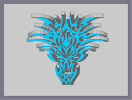Hover over the thumbnail for a full-size version.

Author RavenMac author:ravenmac dragon featured fun-to-look-at n-art nonplayable rated 2010-11-25 2010-11-25 5 by 118 people. \$DRAGON#RavenMac#n-art#00000000000000000000000000000000000000000000000000000000000000000000000000000000000000000000000000000000000000000000000000000000000000000000000000000000000000000000000000000000000000000000000000000000000000000000000000000000000000000000000000000000000000000000000000000000000000000000000000000000000000000000000000000000000000000000000000000000000000000000000000000000000000000000000000000000000000000000000000000000000000000000000000000000000000000000000000000000000000000000000000000000000000000000000000000000000000000000000000000000000000000000000000000000000000000000000000000000000000000000000000000000000000000000000000000000000000000000000000000000000000000000000000000000000000000000000000000000000000000|1^383,500!1^428,500!1^383,499!8^383,499,1!1^385,499!1^405,499!1^428,499!8^428,499,1!1^430,499!1^382,498!8^383,498,1!1^385,498!1^404,498!8^405,498,1!1^408,498!1^428,498!8^428,498,1!1^430,498!1^382,497!8^382,497,1!1^385,497!1^404,497!8^404,497,1!1^409,497!1^428,497!8^428,497,1!1^431,497!1^382,496!8^382,496,1!1^385,496!1^403,496!8^404,496,1!1^409,496!1^428,496!8^428,496,1!1^431,496!1^381,495!8^382,495,1!1^385,495!1^403,495!8^403,495,1!1^410,495!1^428,495!8^428,495,1!1^431,495!1^381,494!8^381,494,1!1^385,494!1^403,494!8^403,494,1!1^410,494!1^428,494!8^428,494,1!1^432,494!1^381,493!8^381,493,1!1^385,493!1^402,493!8^403,493,1!1^410,493!1^428,493!8^428,493,1!1^432,493!1^380,492!8^381,492,1!1^385,492!1^402,492!8^402,492,1!1^411,492!1^428,492!8^428,492,1!1^432,492!1^380,491!8^380,491,1!1^385,491!1^402,491!8^402,491,1!1^411,491!1^428,491!8^428,491,1!1^433,491!1^379,490!8^380,490,1!1^385,490!1^402,490!8^402,490,1!1^411,490!1^428,490!8^428,490,1!1^433,490!1^379,489!8^379,489,1!1^385,489!1^401,489!8^402,489,1!1^411,489!1^428,489!8^428,489,1!1^434,489!1^379,488!8^379,488,1!1^385,488!1^401,488!8^401,488,1!1^412,488!1^428,488!8^428,488,1!1^434,488!1^378,487!8^379,487,1!1^385,487!1^400,487!8^401,487,1!1^412,487!1^428,487!8^428,487,1!1^434,487!1^378,486!8^378,486,1!1^385,486!1^399,486!8^400,486,1!1^413,486!1^428,486!8^428,486,1!1^435,486!1^377,485!8^378,485,1!1^385,485!1^398,485!8^399,485,1!1^414,485!1^428,485!8^428,485,1!1^435,485!1^377,484!8^377,484,1!1^384,484!1^397,484!8^398,484,1!1^415,484!1^428,484!8^429,484,1!1^436,484!1^376,483!8^377,483,1!1^384,483!1^396,483!8^397,483,1!1^406,483!8^407,483,1!1^416,483!1^429,483!8^429,483,1!1^436,483!1^376,482!8^376,482,1!1^384,482!1^394,482!8^396,482,1!1^405,482!8^408,482,1!1^417,482!1^429,482!8^429,482,1!1^437,482!1^375,481!8^376,481,1!1^384,481!1^392,481!8^394,481,1!1^404,481!1^408,481!8^409,481,1!1^419,481!1^429,481!8^429,481,1!1^437,481!1^374,480!8^375,480,1!1^384,480!1^390,480!8^392,480,1!1^404,480!1^409,480!8^409,480,1!1^421,480!1^429,480!8^429,480,1!1^438,480!1^374,479!8^374,479,1!1^383,479!1^389,479!8^390,479,1!1^403,479!1^409,479!8^410,479,1!1^423,479!1^429,479!8^430,479,1!1^439,479!1^373,478!8^374,478,1!1^383,478!1^388,478!8^389,478,1!1^402,478!1^410,478!8^411,478,1!1^424,478!1^430,478!8^430,478,1!1^439,478!1^373,477!8^373,477,1!1^383,477!1^387,477!8^388,477,1!1^402,477!1^411,477!8^411,477,1!1^425,477!1^430,477!8^430,477,1!1^440,477!1^372,476!8^373,476,1!1^383,476!1^386,476!8^387,476,1!1^401,476!1^411,476!8^412,476,1!1^426,476!1^430,476!8^430,476,1!1^440,476!1^372,475!8^372,475,1!1^382,475!1^385,475!8^386,475,1!1^400,475!1^412,475!8^413,475,1!1^427,475!1^430,475!8^431,475,1!1^441,475!1^371,474!8^372,474,1!1^382,474!1^384,474!8^385,474,1!1^400,474!1^413,474!8^413,474,1!1^428,474!1^431,474!8^431,474,1!1^441,474!1^371,473!8^371,473,1!1^382,473!8^384,473,1!1^399,473!1^413,473!8^414,473,1!1^429,473!8^431,473,1!1^442,473!1^370,472!8^371,472,1!1^381,472!8^383,472,1!1^398,472!1^414,472!8^415,472,1!1^430,472!8^432,472,1!1^442,472!1^370,471!8^370,471,1!1^381,471!8^383,471,1!1^398,471!1^415,471!8^415,471,1!1^430,471!8^432,471,1!1^443,471!1^369,470!8^370,470,1!1^381,470!8^382,470,1!1^397,470!1^415,470!8^416,470,1!1^431,470!8^432,470,1!1^443,470!1^368,469!8^369,469,1!1^380,469!8^382,469,1!1^396,469!1^416,469!8^417,469,1!1^431,469!8^433,469,1!1^444,469!1^368,468!8^368,468,1!1^380,468!8^381,468,1!1^396,468!1^417,468!8^417,468,1!1^432,468!8^433,468,1!1^445,468!1^367,467!8^368,467,1!1^379,467!8^381,467,1!1^396,467!1^416,467!8^417,467,1!1^432,467!8^434,467,1!1^445,467!1^366,466!8^367,466,1!1^379,466!8^380,466,1!1^383,466!8^385,466,1!1^397,466!1^415,466!8^416,466,1!1^428,466!8^430,466,1!1^433,466!8^434,466,1!1^446,466!1^366,465!8^366,465,1!1^378,465!1^385,465!8^387,465,1!1^398,465!1^414,465!8^415,465,1!1^426,465!1^430,465!8^435,465,1!1^447,465!1^365,464!8^366,464,1!1^378,464!1^387,464!8^389,464,1!1^399,464!1^413,464!8^414,464,1!1^424,464!1^435,464!8^435,464,1!1^447,464!1^364,463!8^365,463,1!1^377,463!1^389,463!8^390,463,1!1^400,463!1^412,463!8^413,463,1!1^423,463!1^435,463!8^436,463,1!1^448,463!1^364,462!8^364,462,1!1^377,462!1^390,462!8^391,462,1!1^401,462!1^411,462!8^412,462,1!1^422,462!1^436,462!8^436,462,1!1^449,462!1^363,461!8^364,461,1!1^377,461!1^391,461!8^392,461,1!1^402,461!1^410,461!8^411,461,1!1^421,461!1^436,461!8^436,461,1!1^449,461!1^362,460!8^363,460,1!1^376,460!1^392,460!8^392,460,1!1^403,460!1^410,460!8^410,460,1!1^421,460!1^436,460!8^437,460,1!1^450,460!1^362,459!8^362,459,1!1^376,459!1^381,459!1^392,459!8^393,459,1!1^403,459!1^409,459!8^410,459,1!1^420,459!1^429,459!1^437,459!8^437,459,1!1^451,459!1^361,458!8^362,458,1!1^375,458!1^380,458!8^381,458,1!1^384,458!1^393,458!8^394,458,1!1^404,458!1^409,458!8^409,458,1!1^419,458!1^429,458!8^429,458,1!1^432,458!1^437,458!8^438,458,1!1^451,458!1^360,457!8^361,457,1!1^374,457!1^379,457!8^380,457,1!1^384,457!1^394,457!8^394,457,1!1^404,457!1^409,457!8^409,457,1!1^419,457!1^429,457!8^429,457,1!1^433,457!1^438,457!8^439,457,1!1^452,457!1^359,456!8^360,456,1!1^373,456!1^378,456!8^379,456,1!1^383,456!1^394,456!8^394,456,1!1^404,456!1^408,456!8^409,456,1!1^419,456!1^429,456!8^430,456,1!1^434,456!1^439,456!8^440,456,1!1^453,456!1^359,455!8^359,455,1!1^373,455!1^377,455!8^378,455,1!1^382,455!1^394,455!8^395,455,1!1^405,455!1^408,455!8^408,455,1!1^418,455!1^430,455!8^431,455,1!1^435,455!1^440,455!8^440,455,1!1^454,455!1^358,454!8^359,454,1!1^372,454!1^376,454!8^377,454,1!1^382,454!1^395,454!8^395,454,1!1^405,454!1^408,454!8^408,454,1!1^418,454!1^431,454!8^431,454,1!1^436,454!1^440,454!8^441,454,1!1^454,454!1^357,453!8^358,453,1!1^372,453!1^376,453!8^376,453,1!1^381,453!1^395,453!8^395,453,1!1^405,453!1^408,453!8^408,453,1!1^418,453!1^431,453!8^432,453,1!1^437,453!1^441,453!8^441,453,1!1^455,453!1^357,452!8^357,452,1!1^371,452!1^375,452!8^376,452,1!1^381,452!1^395,452!8^395,452,1!1^405,452!1^408,452!8^408,452,1!1^418,452!1^432,452!8^432,452,1!1^437,452!1^441,452!8^442,452,1!1^456,452!1^356,451!8^357,451,1!1^370,451!1^375,451!8^375,451,1!1^381,451!1^395,451!8^395,451,1!1^405,451!1^408,451!8^408,451,1!1^418,451!1^432,451!8^432,451,1!1^438,451!1^442,451!8^443,451,1!1^456,451!1^355,450!8^356,450,1!1^370,450!1^375,450!8^375,450,1!1^381,450!1^395,450!8^395,450,1!1^405,450!1^408,450!8^408,450,1!1^418,450!1^432,450!8^432,450,1!1^438,450!1^443,450!8^443,450,1!1^457,450!1^355,449!8^355,449,1!1^369,449!1^375,449!8^375,449,1!1^380,449!1^395,449!8^395,449,1!1^405,449!1^408,449!8^408,449,1!1^418,449!1^432,449!8^433,449,1!1^438,449!1^443,449!8^444,449,1!1^458,449!1^354,448!8^355,448,1!1^368,448!1^375,448!8^375,448,1!1^381,448!1^394,448!8^395,448,1!1^405,448!1^408,448!8^408,448,1!1^418,448!1^432,448!8^432,448,1!1^438,448!1^444,448!8^445,448,1!1^458,448!1^353,447!8^354,447,1!1^367,447!1^375,447!8^375,447,1!1^381,447!1^394,447!8^394,447,1!1^404,447!1^408,447!8^409,447,1!1^419,447!1^432,447!8^432,447,1!1^438,447!1^445,447!8^446,447,1!1^459,447!1^353,446!8^353,446,1!1^367,446!1^375,446!8^375,446,1!1^381,446!1^393,446!8^394,446,1!1^404,446!1^409,446!8^409,446,1!1^419,446!1^431,446!8^432,446,1!1^438,446!1^446,446!8^446,446,1!1^460,446!1^352,445!8^353,445,1!1^366,445!1^375,445!8^375,445,1!1^382,445!1^392,445!8^393,445,1!1^404,445!1^409,445!8^409,445,1!1^420,445!1^431,445!8^431,445,1!1^438,445!1^446,445!8^447,445,1!1^460,445!1^351,444!8^352,444,1!1^365,444!1^370,444!1^375,444!8^375,444,1!1^382,444!1^391,444!8^392,444,1!1^404,444!1^409,444!8^409,444,1!1^421,444!1^429,444!8^431,444,1!1^438,444!1^441,444!1^447,444!8^448,444,1!1^461,444!1^351,443!8^351,443,1!1^365,443!1^370,443!8^370,443,1!1^372,443!1^375,443!8^375,443,1!1^384,443!1^389,443!8^391,443,1!1^404,443!1^409,443!8^409,443,1!1^422,443!1^427,443!8^429,443,1!1^438,443!1^440,443!8^441,443,1!1^443,443!1^448,443!8^448,443,1!1^462,443!1^350,442!8^351,442,1!1^364,442!1^370,442!8^370,442,1!1^373,442!1^375,442!8^375,442,1!1^386,442!8^389,442,1!1^403,442!1^409,442!8^410,442,1!1^424,442!8^427,442,1!1^438,442!1^440,442!8^440,442,1!1^443,442!1^448,442!8^449,442,1!1^462,442!1^350,441!8^350,441,1!1^364,441!1^370,441!8^370,441,1!1^373,441!8^375,441,1!8^393,441,1!1^403,441!1^410,441!8^410,441,1!8^428,441,1!1^438,441!8^440,441,1!1^443,441!1^449,441!8^449,441,1!1^463,441!1^349,440!8^350,440,1!1^363,440!1^370,440!8^370,440,1!1^374,440!8^376,440,1!8^394,440,1!1^402,440!1^410,440!8^411,440,1!8^429,440,1!1^437,440!8^439,440,1!1^443,440!1^449,440!8^450,440,1!1^463,440!1^349,439!8^349,439,1!1^363,439!1^370,439!8^370,439,1!1^374,439!8^376,439,1!8^394,439,1!1^401,439!1^411,439!8^412,439,1!8^430,439,1!1^437,439!8^439,439,1!1^443,439!1^450,439!8^450,439,1!1^464,439!1^348,438!8^349,438,1!1^363,438!1^370,438!8^371,438,1!1^375,438!8^377,438,1!8^395,438,1!1^401,438!1^412,438!8^412,438,1!8^430,438,1!1^436,438!8^438,438,1!1^442,438!1^450,438!8^450,438,1!1^464,438!1^348,437!8^348,437,1!1^362,437!1^371,437!8^371,437,1!1^375,437!1^377,437!8^378,437,1!8^396,437,1!1^399,437!1^412,437!8^414,437,1!8^432,437,1!1^435,437!1^438,437!8^438,437,1!1^442,437!1^450,437!8^451,437,1!1^465,437!1^347,436!8^348,436,1!1^362,436!1^371,436!8^371,436,1!1^375,436!1^378,436!8^379,436,1!8^397,436,1!1^399,436!1^414,436!8^414,436,1!8^432,436,1!1^434,436!1^437,436!8^438,436,1!1^442,436!1^451,436!8^451,436,1!1^465,436!1^347,435!8^347,435,1!1^362,435!1^370,435!8^371,435,1!1^376,435!1^379,435!8^381,435,1!1^397,435!1^414,435!8^416,435,1!1^432,435!1^437,435!8^437,435,1!1^442,435!1^451,435!8^451,435,1!1^466,435!1^346,434!8^347,434,1!1^362,434!1^370,434!8^370,434,1!1^376,434!1^381,434!8^384,434,1!1^395,434!1^416,434!8^418,434,1!1^429,434!1^436,434!8^437,434,1!1^443,434!1^451,434!8^451,434,1!1^466,434!1^346,433!8^346,433,1!1^362,433!1^370,433!8^370,433,1!1^377,433!1^384,433!1^418,433!1^436,433!8^436,433,1!1^443,433!1^451,433!8^451,433,1!1^467,433!1^346,432!8^346,432,1!1^362,432!1^369,432!8^370,432,1!1^377,432!1^436,432!8^436,432,1!1^443,432!1^450,432!8^451,432,1!1^467,432!1^346,431!8^346,431,1!1^363,431!1^368,431!8^369,431,1!1^377,431!1^435,431!8^436,431,1!1^444,431!1^449,431!8^450,431,1!1^467,431!1^345,430!8^346,430,1!1^364,430!1^367,430!8^368,430,1!1^378,430!1^435,430!8^435,430,1!1^445,430!1^448,430!8^449,430,1!1^467,430!1^345,429!8^345,429,1!8^363,429,1!1^365,429!8^367,429,1!1^378,429!1^435,429!8^435,429,1!1^446,429!8^448,429,1!8^466,429,1!1^468,429!1^345,428!8^345,428,1!8^363,428,1!1^378,428!1^435,428!8^435,428,1!8^453,428,1!1^468,428!1^344,427!8^345,427,1!8^363,427,1!1^378,427!1^434,427!8^435,427,1!8^453,427,1!1^468,427!1^344,426!8^344,426,1!8^362,426,1!1^379,426!1^434,426!8^434,426,1!8^452,426,1!1^469,426!1^344,425!8^344,425,1!8^362,425,1!1^379,425!1^434,425!8^434,425,1!8^452,425,1!1^469,425!1^344,424!8^344,424,1!8^362,424,1!1^379,424!1^398,424!1^413,424!1^434,424!8^434,424,1!8^452,424,1!1^469,424!1^344,423!8^344,423,1!1^357,423!8^361,423,1!1^379,423!1^398,423!8^398,423,1!1^400,423!1^413,423!8^413,423,1!1^415,423!1^434,423!8^434,423,1!1^452,423!8^456,423,1!1^469,423!1^344,422!8^344,422,1!1^355,422!1^361,422!8^363,422,1!1^379,422!1^398,422!8^398,422,1!1^400,422!1^413,422!8^413,422,1!1^415,422!1^434,422!8^434,422,1!1^450,422!1^456,422!8^458,422,1!1^469,422!1^344,421!8^344,421,1!1^354,421!1^363,421!8^364,421,1!1^379,421!1^383,421!1^398,421!8^398,421,1!1^400,421!1^413,421!8^413,421,1!1^415,421!1^428,421!1^434,421!8^434,421,1!1^449,421!1^458,421!8^459,421,1!1^469,421!1^344,420!8^344,420,1!1^353,420!1^364,420!8^365,420,1!1^379,420!1^383,420!8^383,420,1!1^385,420!1^397,420!8^397,420,1!1^399,420!1^413,420!8^414,420,1!1^416,420!1^427,420!8^428,420,1!1^430,420!1^434,420!8^434,420,1!1^448,420!1^459,420!8^460,420,1!1^469,420!1^343,419!8^344,419,1!1^353,419!1^365,419!8^366,419,1!1^379,419!1^383,419!8^384,419,1!1^386,419!1^396,419!8^397,419,1!1^399,419!1^414,419!8^414,419,1!1^416,419!1^426,419!8^427,419,1!1^429,419!1^434,419!8^434,419,1!1^447,419!1^460,419!8^460,419,1!1^469,419!1^343,418!8^343,418,1!1^352,418!1^366,418!8^367,418,1!1^379,418!1^384,418!8^384,418,1!1^387,418!1^396,418!8^396,418,1!1^399,418!1^414,418!8^414,418,1!1^417,418!1^426,418!8^426,418,1!1^429,418!1^434,418!8^434,418,1!1^446,418!1^460,418!8^461,418,1!1^470,418!1^343,417!8^343,417,1!1^352,417!1^367,417!8^367,417,1!1^379,417!1^384,417!8^384,417,1!1^387,417!1^396,417!8^396,417,1!1^399,417!1^414,417!8^414,417,1!1^417,417!1^426,417!8^426,417,1!1^429,417!1^434,417!8^434,417,1!1^446,417!1^461,417!8^461,417,1!1^470,417!1^343,416!8^343,416,1!1^352,416!1^367,416!8^368,416,1!1^379,416!1^384,416!8^385,416,1!1^387,416!1^395,416!8^396,416,1!1^398,416!1^414,416!8^415,416,1!1^417,416!1^425,416!8^426,416,1!1^428,416!1^434,416!8^434,416,1!1^445,416!1^461,416!8^461,416,1!1^470,416!1^343,415!8^343,415,1!1^351,415!1^368,415!8^368,415,1!1^379,415!1^385,415!8^385,415,1!1^388,415!1^395,415!8^395,415,1!1^398,415!1^415,415!8^415,415,1!1^418,415!1^425,415!8^425,415,1!1^428,415!1^434,415!8^434,415,1!1^445,415!1^461,415!8^462,415,1!1^470,415!1^343,414!8^343,414,1!1^351,414!1^368,414!8^368,414,1!1^378,414!1^385,414!8^385,414,1!1^388,414!1^394,414!8^395,414,1!1^398,414!1^415,414!8^415,414,1!1^418,414!1^425,414!8^425,414,1!1^428,414!1^434,414!8^435,414,1!1^445,414!1^462,414!8^462,414,1!1^470,414!1^343,413!8^343,413,1!1^351,413!1^368,413!8^368,413,1!1^378,413!1^385,413!8^385,413,1!1^388,413!1^394,413!8^394,413,1!1^398,413!1^415,413!8^415,413,1!1^419,413!1^424,413!8^425,413,1!1^428,413!1^435,413!8^435,413,1!1^445,413!1^462,413!8^462,413,1!1^470,413!1^343,412!8^344,412,1!1^351,412!1^368,412!8^369,412,1!1^378,412!1^385,412!8^385,412,1!1^389,412!1^394,412!8^394,412,1!1^398,412!1^415,412!8^415,412,1!1^419,412!1^424,412!8^424,412,1!1^428,412!1^435,412!8^435,412,1!1^444,412!1^462,412!8^462,412,1!1^469,412!1^344,411!8^344,411,1!1^351,411!1^369,411!8^369,411,1!1^378,411!1^385,411!8^385,411,1!1^389,411!1^394,411!8^394,411,1!1^398,411!1^415,411!8^415,411,1!1^419,411!1^424,411!8^424,411,1!1^428,411!1^435,411!8^435,411,1!1^444,411!1^462,411!8^462,411,1!1^469,411!1^344,410!8^344,410,1!1^351,410!1^369,410!8^369,410,1!1^378,410!1^385,410!8^385,410,1!1^389,410!1^393,410!8^394,410,1!1^397,410!1^415,410!8^416,410,1!1^419,410!1^424,410!8^424,410,1!1^428,410!1^435,410!8^435,410,1!1^444,410!1^462,410!8^462,410,1!1^469,410!1^344,409!8^344,409,1!1^351,409!1^369,409!8^369,409,1!1^377,409!1^385,409!8^385,409,1!1^389,409!1^393,409!8^393,409,1!1^397,409!1^416,409!8^416,409,1!1^420,409!1^424,409!8^424,409,1!1^428,409!1^435,409!8^436,409,1!1^444,409!1^462,409!8^462,409,1!1^469,409!1^344,408!8^344,408,1!1^351,408!1^369,408!8^369,408,1!1^377,408!1^385,408!8^385,408,1!1^389,408!1^393,408!8^393,408,1!1^397,408!1^416,408!8^416,408,1!1^420,408!1^424,408!8^424,408,1!1^428,408!1^436,408!8^436,408,1!1^444,408!1^462,408!8^462,408,1!1^469,408!1^344,407!8^344,407,1!1^351,407!1^369,407!8^369,407,1!1^377,407!1^385,407!8^385,407,1!1^389,407!1^393,407!8^393,407,1!1^397,407!1^416,407!8^416,407,1!1^420,407!1^424,407!8^424,407,1!1^428,407!1^436,407!8^436,407,1!1^444,407!1^462,407!8^462,407,1!1^469,407!1^344,406!8^345,406,1!1^351,406!1^369,406!8^369,406,1!1^377,406!1^385,406!8^385,406,1!1^389,406!1^393,406!8^393,406,1!1^397,406!1^416,406!8^416,406,1!1^420,406!1^424,406!8^424,406,1!1^428,406!1^436,406!8^436,406,1!1^444,406!1^462,406!8^462,406,1!1^468,406!1^345,405!8^345,405,1!1^351,405!1^368,405!8^369,405,1!1^377,405!1^385,405!8^385,405,1!1^389,405!1^392,405!8^393,405,1!1^397,405!1^416,405!8^416,405,1!1^420,405!1^424,405!8^424,405,1!1^428,405!1^436,405!8^436,405,1!1^444,405!1^462,405!8^462,405,1!1^468,405!1^345,404!8^345,404,1!1^351,404!1^368,404!8^368,404,1!1^377,404!1^385,404!8^385,404,1!1^389,404!1^392,404!8^392,404,1!1^397,404!1^416,404!8^416,404,1!1^421,404!1^424,404!8^424,404,1!1^428,404!1^436,404!8^436,404,1!1^445,404!1^462,404!8^462,404,1!1^468,404!1^345,403!8^346,403,1!1^351,403!1^368,403!8^368,403,1!1^376,403!1^384,403!8^385,403,1!1^389,403!1^392,403!8^392,403,1!1^397,403!1^416,403!8^416,403,1!1^421,403!1^424,403!8^424,403,1!1^428,403!1^436,403!8^437,403,1!1^445,403!1^462,403!8^462,403,1!1^467,403!1^346,402!8^346,402,1!1^351,402!1^368,402!8^368,402,1!1^376,402!1^384,402!8^384,402,1!1^389,402!1^392,402!8^392,402,1!1^397,402!1^416,402!8^416,402,1!1^421,402!1^424,402!8^424,402,1!1^429,402!1^437,402!8^437,402,1!1^445,402!1^461,402!8^462,402,1!1^467,402!1^346,401!8^347,401,1!1^352,401!1^368,401!8^368,401,1!1^376,401!1^383,401!8^384,401,1!1^389,401!1^392,401!8^392,401,1!1^397,401!1^416,401!8^416,401,1!1^421,401!1^424,401!8^424,401,1!1^429,401!1^437,401!8^437,401,1!1^445,401!1^461,401!8^461,401,1!1^466,401!1^347,400!8^347,400,1!1^352,400!1^367,400!8^368,400,1!1^376,400!1^383,400!8^383,400,1!1^389,400!1^392,400!8^392,400,1!1^397,400!1^416,400!8^416,400,1!1^421,400!1^424,400!8^424,400,1!1^430,400!1^437,400!8^437,400,1!1^445,400!1^461,400!8^461,400,1!1^466,400!1^347,399!8^348,399,1!1^352,399!1^367,399!8^367,399,1!1^376,399!1^383,399!8^383,399,1!1^389,399!1^392,399!8^392,399,1!1^397,399!1^416,399!8^416,399,1!1^421,399!1^424,399!8^424,399,1!1^430,399!1^437,399!8^437,399,1!1^446,399!1^460,399!8^461,399,1!1^465,399!1^348,398!8^348,398,1!1^353,398!1^367,398!8^367,398,1!1^375,398!1^382,398!8^383,398,1!1^389,398!1^392,398!8^392,398,1!1^397,398!1^416,398!8^416,398,1!1^421,398!1^424,398!8^424,398,1!1^430,398!1^437,398!8^438,398,1!1^446,398!1^460,398!8^460,398,1!1^465,398!1^348,397!8^349,397,1!1^353,397!1^367,397!8^367,397,1!1^375,397!1^382,397!8^382,397,1!1^389,397!1^391,397!8^392,397,1!1^397,397!1^416,397!8^416,397,1!1^421,397!1^424,397!8^424,397,1!1^431,397!1^438,397!8^438,397,1!1^446,397!1^459,397!8^460,397,1!1^464,397!1^349,396!8^350,396,1!1^354,396!1^366,396!8^367,396,1!1^374,396!1^381,396!8^382,396,1!1^389,396!1^391,396!8^391,396,1!1^397,396!1^416,396!8^416,396,1!1^422,396!1^424,396!8^424,396,1!1^431,396!1^438,396!8^439,396,1!1^446,396!1^459,396!8^459,396,1!1^463,396!1^350,395!1^366,395!8^366,395,1!1^374,395!1^381,395!8^381,395,1!1^388,395!1^391,395!8^391,395,1!1^397,395!1^416,395!8^416,395,1!1^422,395!1^424,395!8^425,395,1!1^432,395!1^439,395!8^439,395,1!1^447,395!1^459,395!1^366,394!8^366,394,1!1^373,394!1^380,394!8^381,394,1!1^388,394!1^391,394!8^391,394,1!1^397,394!1^416,394!8^416,394,1!1^422,394!1^425,394!8^425,394,1!1^432,394!1^439,394!8^440,394,1!1^447,394!1^366,393!8^366,393,1!1^373,393!1^379,393!8^380,393,1!1^388,393!1^391,393!8^391,393,1!1^397,393!1^416,393!8^416,393,1!1^422,393!1^425,393!8^425,393,1!1^433,393!1^440,393!8^440,393,1!1^447,393!1^365,392!8^366,392,1!1^372,392!1^378,392!8^379,392,1!1^388,392!1^391,392!8^391,392,1!1^397,392!1^415,392!8^416,392,1!1^422,392!1^425,392!8^425,392,1!1^434,392!1^440,392!8^441,392,1!1^447,392!1^365,391!8^365,391,1!1^372,391!1^378,391!8^378,391,1!1^388,391!1^391,391!8^391,391,1!1^398,391!1^415,391!8^415,391,1!1^422,391!1^425,391!8^425,391,1!1^435,391!1^441,391!8^441,391,1!1^448,391!1^365,390!8^365,390,1!1^372,390!1^377,390!8^378,390,1!1^388,390!1^391,390!8^391,390,1!1^398,390!1^415,390!8^415,390,1!1^422,390!1^425,390!8^425,390,1!1^435,390!1^441,390!8^441,390,1!1^448,390!1^364,389!8^365,389,1!1^371,389!1^376,389!8^377,389,1!1^387,389!1^391,389!8^391,389,1!1^398,389!1^415,389!8^415,389,1!1^422,389!1^425,389!8^426,389,1!1^436,389!1^441,389!8^442,389,1!1^448,389!1^364,388!8^364,388,1!1^370,388!1^375,388!8^376,388,1!1^387,388!1^391,388!8^391,388,1!1^398,388!1^415,388!8^415,388,1!1^422,388!1^426,388!8^426,388,1!1^437,388!1^442,388!8^443,388,1!1^449,388!1^363,387!8^364,387,1!1^370,387!1^374,387!8^375,387,1!1^387,387!1^391,387!8^391,387,1!1^398,387!1^415,387!8^415,387,1!1^422,387!1^426,387!8^426,387,1!1^438,387!1^443,387!8^443,387,1!1^449,387!1^363,386!8^363,386,1!1^369,386!1^373,386!8^374,386,1!1^386,386!1^391,386!8^391,386,1!1^398,386!1^415,386!8^415,386,1!1^422,386!1^426,386!8^427,386,1!1^439,386!1^443,386!8^444,386,1!1^450,386!1^363,385!8^363,385,1!1^368,385!1^372,385!8^373,385,1!1^386,385!1^391,385!8^391,385,1!1^398,385!1^414,385!8^415,385,1!1^422,385!1^427,385!8^427,385,1!1^440,385!1^444,385!8^445,385,1!1^450,385!1^362,384!8^363,384,1!1^368,384!1^371,384!8^372,384,1!1^386,384!1^391,384!8^391,384,1!1^399,384!1^414,384!8^414,384,1!1^422,384!1^427,384!8^427,384,1!1^441,384!1^445,384!8^445,384,1!1^450,384!1^361,383!8^362,383,1!1^367,383!1^370,383!8^371,383,1!1^386,383!1^391,383!8^391,383,1!1^399,383!1^414,383!8^414,383,1!1^422,383!1^427,383!8^427,383,1!1^442,383!1^445,383!8^446,383,1!1^451,383!1^361,382!8^361,382,1!1^367,382!1^369,382!8^370,382,1!1^385,382!1^391,382!8^391,382,1!1^399,382!1^414,382!8^414,382,1!1^422,382!1^427,382!8^428,382,1!1^443,382!1^446,382!8^446,382,1!1^452,382!1^360,381!8^361,381,1!1^366,381!8^369,381,1!1^385,381!1^391,381!8^391,381,1!1^399,381!1^414,381!8^414,381,1!1^422,381!1^428,381!8^428,381,1!1^444,381!8^447,381,1!1^452,381!1^359,380!8^360,380,1!1^365,380!8^368,380,1!1^384,380!1^391,380!8^391,380,1!1^399,380!1^414,380!8^414,380,1!1^422,380!1^428,380!8^429,380,1!1^445,380!8^448,380,1!1^453,380!1^359,379!8^359,379,1!1^364,379!1^367,379!8^367,379,1!1^384,379!1^391,379!8^391,379,1!1^399,379!1^414,379!8^414,379,1!1^422,379!1^429,379!8^429,379,1!1^446,379!1^448,379!8^449,379,1!1^454,379!1^358,378!8^359,378,1!1^363,378!1^366,378!8^367,378,1!1^383,378!1^391,378!8^392,378,1!1^399,378!1^413,378!8^414,378,1!1^421,378!1^429,378!8^430,378,1!1^446,378!1^449,378!8^450,378,1!1^454,378!1^357,377!8^358,377,1!1^362,377!1^365,377!8^366,377,1!1^383,377!1^392,377!8^392,377,1!1^400,377!1^413,377!8^413,377,1!1^421,377!1^430,377!8^430,377,1!1^447,377!1^450,377!8^451,377,1!1^455,377!1^356,376!8^357,376,1!1^362,376!1^365,376!8^365,376,1!1^382,376!1^392,376!8^392,376,1!1^400,376!1^413,376!8^413,376,1!1^421,376!1^430,376!8^431,376,1!1^448,376!1^451,376!8^451,376,1!1^456,376!1^355,375!8^356,375,1!1^361,375!1^364,375!8^365,375,1!1^382,375!1^392,375!8^392,375,1!1^400,375!1^413,375!8^413,375,1!1^421,375!1^431,375!8^431,375,1!1^448,375!1^451,375!8^452,375,1!1^457,375!1^354,374!8^355,374,1!1^359,374!1^364,374!8^364,374,1!1^381,374!1^392,374!8^392,374,1!1^400,374!1^412,374!8^413,374,1!1^421,374!1^431,374!8^432,374,1!1^449,374!1^452,374!8^454,374,1!1^458,374!1^353,373!8^354,373,1!1^358,373!1^363,373!8^364,373,1!1^381,373!1^392,373!8^392,373,1!1^401,373!1^412,373!8^412,373,1!1^421,373!1^432,373!8^432,373,1!1^449,373!1^454,373!8^455,373,1!1^459,373!1^352,372!8^353,372,1!1^357,372!1^363,372!8^363,372,1!1^380,372!1^392,372!8^393,372,1!1^401,372!1^412,372!8^412,372,1!1^420,372!1^432,372!8^433,372,1!1^450,372!1^455,372!8^456,372,1!1^460,372!1^352,371!8^352,371,1!1^356,371!1^362,371!8^363,371,1!1^368,371!8^370,371,1!1^380,371!1^393,371!8^393,371,1!1^401,371!1^411,371!8^412,371,1!1^420,371!1^433,371!8^433,371,1!1^443,371!8^445,371,1!1^450,371!1^456,371!8^457,371,1!1^461,371!1^352,370!1^362,370!8^362,370,1!1^367,370!1^371,370!8^372,370,1!1^380,370!1^393,370!8^393,370,1!1^402,370!1^411,370!8^411,370,1!1^420,370!1^433,370!8^433,370,1!1^441,370!1^445,370!8^446,370,1!1^451,370!1^457,370!1^361,369!8^362,369,1!1^365,369!1^372,369!8^372,369,1!1^379,369!1^393,369!8^393,369,1!1^402,369!1^410,369!8^411,369,1!1^420,369!1^433,369!8^434,369,1!1^441,369!1^446,369!8^448,369,1!1^451,369!1^361,368!8^361,368,1!1^364,368!1^372,368!8^373,368,1!1^379,368!1^393,368!8^393,368,1!1^403,368!1^409,368!8^410,368,1!1^420,368!1^434,368!8^434,368,1!1^440,368!1^448,368!8^449,368,1!1^452,368!1^360,367!8^361,367,1!1^363,367!1^373,367!8^373,367,1!1^379,367!1^393,367!8^394,367,1!1^404,367!1^408,367!8^409,367,1!1^419,367!1^434,367!8^434,367,1!1^440,367!1^449,367!8^450,367,1!1^452,367!1^360,366!8^360,366,1!1^362,366!1^373,366!8^373,366,1!1^379,366!1^394,366!8^394,366,1!1^405,366!8^408,366,1!1^419,366!1^434,366!8^434,366,1!1^440,366!1^450,366!8^451,366,1!1^453,366!1^360,365!8^360,365,1!1^362,365!1^372,365!8^373,365,1!1^379,365!1^388,365!1^394,365!8^394,365,1!8^412,365,1!1^419,365!1^423,365!1^434,365!8^434,365,1!1^440,365!1^451,365!8^451,365,1!1^453,365!1^348,364!1^360,364!1^372,364!8^372,364,1!1^379,364!1^388,364!8^388,364,1!1^390,364!1^394,364!8^395,364,1!8^413,364,1!1^418,364!1^423,364!8^423,364,1!1^425,364!1^434,364!8^434,364,1!1^441,364!1^451,364!1^457,364!1^344,363!8^348,363,1!1^356,363!1^371,363!8^372,363,1!1^379,363!1^387,363!8^388,363,1!1^390,363!1^395,363!8^395,363,1!8^413,363,1!1^418,363!1^423,363!8^423,363,1!1^425,363!1^434,363!8^434,363,1!1^441,363!1^457,363!8^457,363,1!1^465,363!1^340,362!8^344,362,1!1^355,362!1^370,362!8^371,362,1!1^379,362!1^386,362!8^387,362,1!1^390,362!1^395,362!8^396,362,1!8^414,362,1!1^417,362!1^423,362!8^423,362,1!1^426,362!1^433,362!8^434,362,1!1^442,362!1^457,362!8^458,362,1!1^469,362!1^337,361!8^340,361,1!1^354,361!1^369,361!8^370,361,1!1^380,361!1^385,361!8^386,361,1!1^390,361!1^396,361!8^396,361,1!8^414,361,1!1^417,361!1^423,361!8^423,361,1!1^427,361!1^432,361!8^433,361,1!1^443,361!1^458,361!8^459,361,1!1^473,361!1^335,360!8^337,360,1!1^352,360!1^368,360!8^369,360,1!1^381,360!8^385,360,1!1^390,360!1^396,360!8^397,360,1!1^416,360!1^423,360!8^423,360,1!1^428,360!8^432,360,1!1^444,360!1^459,360!8^461,360,1!1^476,360!1^332,359!8^335,359,1!1^350,359!1^367,359!8^368,359,1!1^383,359!8^383,359,1!1^390,359!1^397,359!8^397,359,1!1^416,359!1^423,359!8^423,359,1!1^430,359!8^430,359,1!1^445,359!1^461,359!8^463,359,1!1^478,359!1^330,358!8^332,358,1!1^349,358!1^366,358!8^367,358,1!8^385,358,1!1^390,358!1^397,358!8^398,358,1!1^415,358!1^423,358!8^423,358,1!8^441,358,1!1^446,358!1^463,358!8^464,358,1!1^481,358!1^328,357!8^330,357,1!1^348,357!1^365,357!8^366,357,1!8^384,357,1!1^390,357!1^398,357!8^399,357,1!1^414,357!1^423,357!8^423,357,1!8^441,357,1!1^447,357!1^464,357!8^465,357,1!1^483,357!1^326,356!8^328,356,1!1^347,356!1^364,356!8^365,356,1!8^383,356,1!1^390,356!1^399,356!8^400,356,1!1^413,356!1^423,356!8^423,356,1!8^441,356,1!1^448,356!1^465,356!8^466,356,1!1^485,356!1^324,355!8^326,355,1!8^344,355,1!1^346,355!1^363,355!8^364,355,1!8^382,355,1!1^389,355!1^400,355!8^400,355,1!1^413,355!1^423,355!8^424,355,1!8^442,355,1!1^449,355!1^466,355!8^467,355,1!8^485,355,1!1^487,355!1^322,354!8^324,354,1!8^342,354,1!1^345,354!1^362,354!8^363,354,1!8^381,354,1!1^389,354!1^399,354!8^400,354,1!1^413,354!1^424,354!8^424,354,1!8^442,354,1!1^450,354!1^467,354!8^468,354,1!8^486,354,1!1^489,354!1^321,353!8^322,353,1!8^340,353,1!1^343,353!1^361,353!8^362,353,1!8^380,353,1!1^388,353!1^398,353!8^399,353,1!1^414,353!1^424,353!8^425,353,1!8^443,353,1!1^451,353!1^468,353!8^470,353,1!8^488,353,1!1^491,353!1^319,352!8^321,352,1!8^339,352,1!1^342,352!1^359,352!8^361,352,1!8^379,352,1!1^388,352!1^398,352!8^398,352,1!1^415,352!1^425,352!8^425,352,1!8^443,352,1!1^452,352!1^470,352!8^471,352,1!8^489,352,1!1^492,352!1^318,351!8^319,351,1!8^337,351,1!1^341,351!1^359,351!8^359,351,1!8^377,351,1!1^387,351!1^397,351!8^398,351,1!1^415,351!1^425,351!8^426,351,1!8^444,351,1!1^454,351!1^471,351!8^472,351,1!8^490,351,1!1^494,351!1^317,350!8^318,350,1!8^336,350,1!1^340,350!1^358,350!8^359,350,1!1^366,350!8^371,350,1!1^386,350!1^396,350!8^397,350,1!1^416,350!1^426,350!8^427,350,1!1^442,350!8^447,350,1!1^454,350!1^472,350!8^473,350,1!8^491,350,1!1^495,350!1^315,349!8^317,349,1!8^335,349,1!1^339,349!1^357,349!8^358,349,1!1^364,349!1^371,349!8^372,349,1!1^386,349!1^395,349!8^396,349,1!8^414,349,1!1^417,349!1^427,349!8^427,349,1!1^441,349!1^447,349!8^449,349,1!1^455,349!1^473,349!8^474,349,1!8^492,349,1!1^496,349!1^314,348!8^315,348,1!8^333,348,1!1^338,348!1^356,348!8^357,348,1!1^363,348!1^372,348!8^373,348,1!1^385,348!1^394,348!8^395,348,1!8^413,348,1!1^418,348!1^427,348!8^428,348,1!1^440,348!1^449,348!8^450,348,1!1^456,348!1^474,348!8^475,348,1!8^493,348,1!1^498,348!1^312,347!8^314,347,1!8^332,347,1!1^337,347!1^355,347!8^356,347,1!1^361,347!1^373,347!8^374,347,1!1^384,347!1^393,347!8^394,347,1!8^412,347,1!1^419,347!1^428,347!8^429,347,1!1^439,347!1^450,347!8^452,347,1!1^457,347!1^475,347!8^476,347,1!8^494,347,1!1^499,347!1^311,346!8^312,346,1!8^330,346,1!1^336,346!1^355,346!8^355,346,1!1^360,346!1^373,346!8^374,346,1!1^383,346!1^392,346!8^393,346,1!8^411,346,1!1^420,346!1^429,346!8^430,346,1!1^439,346!1^452,346!8^453,346,1!1^458,346!1^476,346!8^477,346,1!8^495,346,1!1^501,346!1^310,345!8^311,345,1!8^329,345,1!1^335,345!1^355,345!8^355,345,1!1^359,345!1^373,345!8^373,345,1!1^382,345!1^391,345!8^392,345,1!8^410,345,1!1^421,345!1^430,345!8^431,345,1!1^440,345!1^453,345!8^454,345,1!1^458,345!1^477,345!8^478,345,1!8^496,345,1!1^502,345!1^309,344!8^310,344,1!8^328,344,1!1^334,344!1^354,344!8^355,344,1!1^358,344!1^373,344!8^373,344,1!1^381,344!1^390,344!8^391,344,1!8^409,344,1!1^422,344!1^431,344!8^432,344,1!1^440,344!1^454,344!8^455,344,1!1^458,344!1^478,344!8^479,344,1!8^497,344,1!1^503,344!1^308,343!8^309,343,1!8^327,343,1!1^333,343!1^354,343!8^354,343,1!1^357,343!1^372,343!8^373,343,1!1^379,343!1^388,343!8^390,343,1!8^408,343,1!1^423,343!1^432,343!8^434,343,1!1^440,343!1^455,343!8^456,343,1!1^459,343!1^479,343!8^480,343,1!8^498,343,1!1^504,343!1^307,342!8^308,342,1!8^326,342,1!1^332,342!1^353,342!8^354,342,1!1^357,342!1^371,342!8^372,342,1!1^378,342!1^387,342!8^388,342,1!8^406,342,1!1^425,342!1^434,342!8^435,342,1!1^441,342!1^456,342!8^456,342,1!1^459,342!1^480,342!8^481,342,1!8^499,342,1!1^505,342!1^307,341!8^307,341,1!8^325,341,1!1^331,341!1^353,341!8^353,341,1!1^356,341!1^369,341!8^371,341,1!1^375,341!1^386,341!8^387,341,1!1^404,341!8^409,341,1!1^426,341!1^435,341!8^438,341,1!1^442,341!1^456,341!8^457,341,1!1^460,341!1^481,341!8^482,341,1!8^500,341,1!1^506,341!1^306,340!8^307,340,1!8^325,340,1!1^330,340!1^353,340!8^353,340,1!1^355,340!1^369,340!8^369,340,1!1^373,340!1^385,340!8^386,340,1!1^402,340!1^409,340!8^411,340,1!1^427,340!1^438,340!8^440,340,1!1^444,340!1^457,340!8^458,340,1!1^460,340!1^482,340!8^483,340,1!8^501,340,1!1^506,340!1^305,339!8^306,339,1!8^324,339,1!1^330,339!1^353,339!8^353,339,1!1^355,339!1^369,339!1^384,339!8^385,339,1!1^400,339!1^411,339!8^413,339,1!1^428,339!1^440,339!1^458,339!8^458,339,1!1^460,339!1^483,339!8^483,339,1!8^501,339,1!1^507,339!1^304,338!8^305,338,1!8^323,338,1!1^329,338!1^353,338!1^382,338!8^384,338,1!1^398,338!1^413,338!8^415,338,1!1^429,338!1^458,338!1^483,338!8^484,338,1!8^502,338,1!1^508,338!1^303,337!8^304,337,1!8^322,337,1!1^328,337!1^380,337!8^382,337,1!1^397,337!1^415,337!8^416,337,1!1^431,337!1^484,337!8^485,337,1!8^503,337,1!1^509,337!1^303,336!8^303,336,1!8^321,336,1!1^327,336!1^377,336!8^380,336,1!1^395,336!1^416,336!8^418,336,1!1^433,336!1^485,336!8^486,336,1!8^504,336,1!1^510,336!1^302,335!8^303,335,1!8^321,335,1!1^326,335!1^375,335!8^377,335,1!1^394,335!1^418,335!8^419,335,1!1^436,335!1^486,335!8^487,335,1!8^505,335,1!1^510,335!1^301,334!8^302,334,1!8^320,334,1!1^325,334!1^372,334!8^375,334,1!1^393,334!1^419,334!8^420,334,1!1^438,334!1^487,334!8^488,334,1!8^506,334,1!1^511,334!1^300,333!8^301,333,1!8^319,333,1!1^325,333!1^364,333!8^372,333,1!1^391,333!1^420,333!8^422,333,1!1^441,333!1^488,333!8^488,333,1!8^506,333,1!1^512,333!1^299,332!8^300,332,1!8^318,332,1!1^324,332!1^360,332!8^364,332,1!8^382,332,1!1^390,332!1^422,332!8^423,332,1!8^441,332,1!1^449,332!1^488,332!8^489,332,1!8^507,332,1!1^513,332!1^299,331!8^299,331,1!8^317,331,1!1^323,331!1^358,331!8^360,331,1!8^378,331,1!1^389,331!1^423,331!8^424,331,1!8^442,331,1!1^453,331!1^489,331!8^490,331,1!8^508,331,1!1^514,331!1^298,330!8^299,330,1!8^317,330,1!1^323,330!1^355,330!8^358,330,1!8^376,330,1!1^388,330!1^424,330!8^425,330,1!8^443,330,1!1^455,330!1^490,330!8^490,330,1!8^508,330,1!1^514,330!1^298,329!8^298,329,1!8^316,329,1!1^322,329!1^353,329!8^355,329,1!8^373,329,1!1^387,329!1^425,329!8^426,329,1!8^444,329,1!1^458,329!1^490,329!8^491,329,1!8^509,329,1!1^515,329!1^297,328!8^298,328,1!8^316,328,1!1^321,328!1^352,328!8^353,328,1!8^371,328,1!1^386,328!1^426,328!8^427,328,1!8^445,328,1!1^460,328!1^491,328!8^492,328,1!8^510,328,1!1^515,328!1^296,327!8^297,327,1!8^315,327,1!1^321,327!1^350,327!8^352,327,1!8^370,327,1!1^385,327!1^427,327!8^428,327,1!8^446,327,1!1^461,327!1^492,327!8^492,327,1!8^510,327,1!1^516,327!1^296,326!8^296,326,1!8^314,326,1!1^320,326!1^349,326!8^350,326,1!8^368,326,1!1^384,326!1^428,326!8^429,326,1!8^447,326,1!1^463,326!1^492,326!8^493,326,1!8^511,326,1!1^517,326!1^295,325!8^296,325,1!8^314,325,1!1^320,325!1^347,325!8^349,325,1!1^364,325!8^371,325,1!1^383,325!1^429,325!8^430,325,1!1^442,325!8^449,325,1!1^464,325!1^493,325!8^493,325,1!8^511,325,1!1^517,325!1^295,324!8^295,324,1!8^313,324,1!1^320,324!1^346,324!8^347,324,1!1^360,324!1^371,324!8^373,324,1!1^382,324!1^405,324!1^430,324!8^431,324,1!1^440,324!1^449,324!8^453,324,1!1^466,324!1^493,324!8^493,324,1!8^511,324,1!1^518,324!1^294,323!8^295,323,1!8^313,323,1!1^319,323!1^345,323!8^346,323,1!1^359,323!1^372,323!8^373,323,1!1^381,323!1^405,323!8^405,323,1!1^408,323!1^431,323!8^432,323,1!1^440,323!1^453,323!8^454,323,1!1^467,323!1^493,323!8^494,323,1!8^512,323,1!1^518,323!1^294,322!8^294,322,1!8^312,322,1!1^319,322!1^344,322!8^345,322,1!1^357,322!1^371,322!8^372,322,1!1^380,322!1^405,322!8^405,322,1!1^408,322!1^432,322!8^433,322,1!1^441,322!1^454,322!8^456,322,1!1^468,322!1^494,322!8^494,322,1!8^512,322,1!1^519,322!1^294,321!8^294,321,1!8^312,321,1!1^318,321!1^344,321!8^344,321,1!1^356,321!1^371,321!8^371,321,1!1^379,321!1^395,321!1^405,321!8^405,321,1!1^408,321!1^416,321!1^433,321!8^434,321,1!1^442,321!1^456,321!8^457,321,1!1^469,321!1^494,321!8^495,321,1!8^513,321,1!1^519,321!1^293,320!8^294,320,1!8^312,320,1!1^318,320!1^343,320!8^344,320,1!1^355,320!1^370,320!8^371,320,1!1^378,320!1^393,320!8^395,320,1!1^397,320!1^405,320!8^405,320,1!1^408,320!1^416,320!8^416,320,1!1^418,320!1^434,320!8^435,320,1!1^442,320!1^457,320!8^458,320,1!1^469,320!1^495,320!8^495,320,1!8^513,320,1!1^519,320!1^293,319!8^293,319,1!8^311,319,1!1^318,319!1^343,319!8^343,319,1!1^353,319!1^369,319!8^370,319,1!1^378,319!1^391,319!8^393,319,1!1^396,319!1^405,319!8^405,319,1!1^408,319!1^416,319!8^417,319,1!1^420,319!1^435,319!8^435,319,1!1^443,319!1^458,319!8^460,319,1!1^470,319!1^495,319!8^495,319,1!8^513,319,1!1^520,319!1^293,318!8^293,318,1!1^298,318!8^303,318,1!1^318,318!1^342,318!8^343,318,1!1^352,318!1^369,318!8^369,318,1!1^377,318!1^389,318!8^391,318,1!1^395,318!1^404,318!8^405,318,1!1^408,318!1^417,318!8^418,318,1!1^422,318!1^435,318!8^436,318,1!1^444,318!1^460,318!8^461,318,1!1^470,318!1^495,318!8^495,318,1!1^510,318!8^515,318,1!1^520,318!1^293,317!8^293,317,1!1^296,317!1^303,317!8^305,317,1!1^318,317!1^342,317!8^342,317,1!1^351,317!1^368,317!8^369,317,1!1^376,317!1^388,317!8^389,317,1!1^394,317!1^404,317!8^404,317,1!1^409,317!1^418,317!8^419,317,1!1^424,317!1^436,317!8^437,317,1!1^444,317!1^461,317!8^462,317,1!1^471,317!1^495,317!8^495,317,1!1^508,317!1^515,317!8^517,317,1!1^520,317!1^293,316!8^293,316,1!1^295,316!1^305,316!8^306,316,1!1^318,316!1^341,316!8^342,316,1!1^350,316!1^367,316!8^368,316,1!1^375,316!1^386,316!8^388,316,1!1^393,316!1^404,316!8^404,316,1!1^409,316!1^419,316!8^420,316,1!1^425,316!1^437,316!8^438,316,1!1^445,316!1^462,316!8^463,316,1!1^471,316!1^495,316!8^495,316,1!1^507,316!1^517,316!8^518,316,1!1^520,316!1^293,315!1^306,315!8^307,315,1!1^318,315!1^331,315!1^341,315!8^341,315,1!1^350,315!1^366,315!8^367,315,1!1^374,315!1^385,315!8^386,315,1!1^392,315!1^404,315!8^404,315,1!1^409,315!1^420,315!8^421,315,1!1^427,315!1^438,315!8^439,315,1!1^446,315!1^463,315!8^463,315,1!1^472,315!1^480,315!1^494,315!8^495,315,1!1^506,315!1^518,315!1^307,314!8^308,314,1!1^319,314!1^330,314!8^331,314,1!1^333,314!1^341,314!8^341,314,1!1^349,314!1^358,314!8^366,314,1!1^373,314!1^384,314!8^385,314,1!1^391,314!1^404,314!8^404,314,1!1^409,314!1^421,314!8^422,314,1!1^428,314!1^439,314!8^440,314,1!1^447,314!1^463,314!8^464,314,1!1^472,314!1^480,314!8^480,314,1!1^482,314!1^493,314!8^494,314,1!1^505,314!1^308,313!8^308,313,1!1^320,313!1^328,313!8^330,313,1!1^333,313!1^340,313!8^341,313,1!1^349,313!1^356,313!8^358,313,1!1^373,313!1^382,313!8^384,313,1!1^390,313!1^404,313!8^404,313,1!1^409,313!1^422,313!8^423,313,1!1^429,313!1^440,313!8^440,313,1!1^455,313!1^464,313!8^464,313,1!1^472,313!1^480,313!8^480,313,1!1^483,313!1^492,313!8^493,313,1!1^505,313!1^308,312!8^309,312,1!1^321,312!8^328,312,1!1^333,312!1^340,312!8^340,312,1!1^348,312!1^355,312!8^356,312,1!1^372,312!1^381,312!8^382,312,1!1^389,312!1^403,312!8^404,312,1!1^409,312!1^423,312!8^424,312,1!1^431,312!1^440,312!8^441,312,1!1^457,312!1^464,312!8^465,312,1!1^473,312!1^480,312!8^480,312,1!1^485,312!8^492,312,1!1^504,312!1^309,311!8^309,311,1!8^327,311,1!1^332,311!1^340,311!8^340,311,1!1^348,311!1^354,311!8^355,311,1!1^371,311!1^380,311!8^381,311,1!1^388,311!1^403,311!8^403,311,1!1^410,311!1^424,311!8^425,311,1!1^432,311!1^441,311!8^442,311,1!1^458,311!1^465,311!8^465,311,1!1^473,311!1^480,311!8^481,311,1!8^499,311,1!1^504,311!1^309,310!8^309,310,1!8^327,310,1!1^332,310!1^339,310!8^340,310,1!1^347,310!1^354,310!8^354,310,1!1^370,310!1^379,310!8^380,310,1!1^388,310!1^403,310!8^403,310,1!1^410,310!1^425,310!8^425,310,1!1^433,310!1^442,310!8^443,310,1!1^459,310!1^465,310!8^466,310,1!1^473,310!1^481,310!8^481,310,1!8^499,310,1!1^504,310!1^309,309!8^309,309,1!8^327,309,1!1^331,309!1^339,309!8^339,309,1!1^347,309!1^353,309!8^354,309,1!1^370,309!1^378,309!8^379,309,1!1^387,309!1^402,309!8^403,309,1!1^410,309!1^425,309!8^426,309,1!1^434,309!1^443,309!8^443,309,1!1^459,309!1^466,309!8^466,309,1!1^474,309!1^481,309!8^482,309,1!8^500,309,1!1^504,309!1^309,308!8^309,308,1!8^327,308,1!1^331,308!1^339,308!8^339,308,1!1^346,308!1^353,308!8^353,308,1!1^369,308!1^377,308!8^378,308,1!1^386,308!1^402,308!8^402,308,1!1^411,308!1^426,308!8^427,308,1!1^435,308!1^443,308!8^444,308,1!1^460,308!1^466,308!8^467,308,1!1^474,308!1^482,308!8^482,308,1!8^500,308,1!1^504,308!1^309,307!8^309,307,1!8^327,307,1!1^330,307!1^339,307!8^339,307,1!1^346,307!1^353,307!8^353,307,1!1^368,307!1^376,307!8^377,307,1!1^385,307!1^402,307!8^402,307,1!1^411,307!1^427,307!8^428,307,1!1^436,307!1^444,307!8^445,307,1!1^460,307!1^467,307!8^467,307,1!1^474,307!1^482,307!8^483,307,1!8^501,307,1!1^504,307!1^308,306!8^309,306,1!8^327,306,1!1^330,306!1^339,306!8^339,306,1!1^346,306!1^353,306!8^353,306,1!1^367,306!1^375,306!8^376,306,1!1^384,306!1^402,306!8^402,306,1!1^411,306!1^428,306!8^429,306,1!1^437,306!1^445,306!8^446,306,1!1^460,306!1^467,306!8^467,306,1!1^474,306!1^483,306!8^483,306,1!8^501,306,1!1^504,306!1^308,305!8^308,305,1!8^326,305,1!1^329,305!1^338,305!8^339,305,1!1^345,305!1^353,305!8^353,305,1!1^367,305!1^374,305!8^375,305,1!1^384,305!1^401,305!8^402,305,1!1^411,305!1^429,305!8^429,305,1!1^438,305!1^446,305!8^446,305,1!1^460,305!1^467,305!8^468,305,1!1^474,305!1^483,305!8^484,305,1!8^502,305,1!1^505,305!1^307,304!8^308,304,1!8^326,304,1!1^329,304!1^338,304!8^338,304,1!1^345,304!1^353,304!8^353,304,1!1^366,304!1^373,304!8^374,304,1!1^383,304!1^401,304!8^401,304,1!1^412,304!1^429,304!8^430,304,1!1^439,304!1^446,304!8^447,304,1!1^460,304!1^468,304!8^468,304,1!1^475,304!1^484,304!8^484,304,1!8^502,304,1!1^505,304!1^307,303!8^307,303,1!8^325,303,1!1^328,303!1^338,303!8^338,303,1!1^345,303!1^353,303!8^353,303,1!1^365,303!1^372,303!8^373,303,1!1^382,303!1^401,303!8^401,303,1!1^412,303!1^430,303!8^431,303,1!1^440,303!1^447,303!8^448,303,1!1^460,303!1^468,303!8^468,303,1!1^475,303!1^484,303!8^485,303,1!8^503,303,1!1^506,303!1^306,302!8^307,302,1!8^325,302,1!1^327,302!1^338,302!8^338,302,1!1^345,302!1^353,302!8^353,302,1!1^364,302!1^371,302!8^372,302,1!1^382,302!1^400,302!8^401,302,1!1^412,302!1^431,302!8^431,302,1!1^441,302!1^448,302!8^449,302,1!1^460,302!1^468,302!8^468,302,1!1^475,302!1^485,302!8^486,302,1!8^504,302,1!1^506,302!1^305,301!8^306,301,1!8^324,301,1!1^327,301!1^338,301!8^338,301,1!1^345,301!1^353,301!8^353,301,1!1^363,301!1^370,301!8^371,301,1!1^381,301!1^400,301!8^400,301,1!1^413,301!1^431,301!8^432,301,1!1^442,301!1^449,301!8^450,301,1!1^460,301!1^467,301!8^468,301,1!1^475,301!1^486,301!8^486,301,1!8^504,301,1!1^507,301!1^304,300!8^305,300,1!8^323,300,1!1^326,300!1^338,300!8^338,300,1!1^346,300!1^353,300!8^354,300,1!1^363,300!1^369,300!8^370,300,1!1^380,300!1^400,300!8^400,300,1!1^413,300!1^432,300!8^433,300,1!1^443,300!1^450,300!8^450,300,1!1^459,300!1^467,300!8^467,300,1!1^475,300!1^486,300!8^487,300,1!8^505,300,1!1^508,300!1^246,299!1^302,299!8^304,299,1!8^322,299,1!1^325,299!1^338,299!8^338,299,1!1^346,299!1^353,299!8^354,299,1!1^362,299!1^368,299!8^369,299,1!1^380,299!1^399,299!8^400,299,1!1^413,299!1^433,299!8^433,299,1!1^444,299!1^450,299!8^451,299,1!1^459,299!1^467,299!8^467,299,1!1^475,299!1^487,299!8^488,299,1!8^506,299,1!1^509,299!1^563,299!1^246,298!8^246,298,1!1^250,298!1^301,298!8^302,298,1!8^320,298,1!1^324,298!1^338,298!8^338,298,1!1^346,298!1^352,298!8^353,298,1!1^361,298!1^367,298!8^368,298,1!1^379,298!1^399,298!8^399,298,1!1^414,298!1^433,298!8^434,298,1!1^445,298!1^451,298!8^452,298,1!1^460,298!1^467,298!8^467,298,1!1^475,298!1^488,298!8^489,298,1!8^507,298,1!1^511,298!1^561,298!8^563,298,1!1^567,298!1^246,297!8^247,297,1!1^252,297!1^299,297!8^301,297,1!8^319,297,1!1^323,297!1^337,297!8^338,297,1!1^346,297!1^350,297!8^352,297,1!1^361,297!1^366,297!8^367,297,1!1^379,297!1^398,297!8^399,297,1!1^414,297!1^434,297!8^434,297,1!1^446,297!1^452,297!8^452,297,1!1^461,297!1^467,297!8^467,297,1!1^475,297!1^489,297!8^490,297,1!8^508,297,1!1^512,297!1^559,297!8^561,297,1!1^566,297!1^247,296!8^249,296,1!1^254,296!1^296,296!8^299,296,1!8^317,296,1!1^323,296!1^337,296!8^337,296,1!1^346,296!1^349,296!8^350,296,1!1^360,296!1^365,296!8^366,296,1!1^378,296!1^398,296!8^398,296,1!1^415,296!1^434,296!8^435,296,1!1^447,296!1^452,296!8^453,296,1!1^463,296!1^466,296!8^467,296,1!1^476,296!1^490,296!8^490,296,1!8^508,296,1!1^514,296!1^556,296!8^559,296,1!1^564,296!1^249,295!8^250,295,1!1^257,295!1^294,295!8^296,295,1!8^314,295,1!1^322,295!1^337,295!8^337,295,1!1^347,295!8^349,295,1!1^359,295!1^364,295!8^365,295,1!1^378,295!1^398,295!8^398,295,1!1^415,295!1^435,295!8^435,295,1!1^448,295!1^453,295!8^454,295,1!1^464,295!8^466,295,1!1^476,295!1^490,295!8^491,295,1!8^509,295,1!1^517,295!1^553,295!8^556,295,1!1^563,295!1^250,294!8^251,294,1!1^260,294!1^292,294!8^294,294,1!8^312,294,1!1^321,294!1^337,294!8^337,294,1!1^347,294!8^348,294,1!1^358,294!1^363,294!8^364,294,1!1^378,294!1^397,294!8^398,294,1!1^415,294!1^435,294!8^435,294,1!1^449,294!1^454,294!8^455,294,1!1^465,294!8^466,294,1!1^476,294!1^491,294!8^492,294,1!8^510,294,1!1^519,294!1^549,294!8^553,294,1!1^562,294!1^251,293!8^253,293,1!1^264,293!1^288,293!8^292,293,1!8^310,293,1!1^320,293!1^336,293!8^337,293,1!8^355,293,1!1^357,293!1^362,293!8^363,293,1!1^377,293!1^396,293!8^397,293,1!1^416,293!1^435,293!8^436,293,1!1^450,293!1^455,293!8^456,293,1!8^474,293,1!1^476,293!1^492,293!8^493,293,1!8^511,293,1!1^521,293!1^544,293!8^549,293,1!1^560,293!1^253,292!8^255,292,1!1^269,292!1^283,292!8^288,292,1!8^306,292,1!1^319,292!1^335,292!8^336,292,1!8^354,292,1!1^356,292!1^362,292!8^362,292,1!1^377,292!1^396,292!8^396,292,1!8^414,292,1!1^417,292!1^436,292!8^436,292,1!1^451,292!1^456,292!8^457,292,1!8^475,292,1!1^477,292!1^493,292!8^494,292,1!8^512,292,1!1^525,292!1^538,292!8^544,292,1!1^558,292!1^255,291!8^256,291,1!1^275,291!8^283,291,1!8^301,291,1!1^318,291!1^335,291!8^335,291,1!8^353,291,1!1^355,291!1^361,291!8^362,291,1!1^377,291!1^395,291!8^396,291,1!8^414,291,1!1^417,291!1^436,291!8^436,291,1!1^451,291!1^457,291!8^458,291,1!8^476,291,1!1^478,291!1^494,291!8^495,291,1!8^513,291,1!1^530,291!8^538,291,1!1^557,291!1^256,290!8^258,290,1!8^276,290,1!8^294,290,1!8^312,290,1!1^317,290!1^334,290!8^335,290,1!1^354,290!1^360,290!8^361,290,1!1^377,290!1^395,290!8^395,290,1!8^413,290,1!1^418,290!1^436,290!8^436,290,1!1^452,290!1^458,290!8^459,290,1!1^478,290!1^495,290!8^496,290,1!8^514,290,1!8^532,290,1!8^550,290,1!1^555,290!1^258,289!8^260,289,1!8^278,289,1!8^296,289,1!1^315,289!1^332,289!8^334,289,1!1^353,289!1^359,289!8^360,289,1!1^376,289!1^395,289!8^395,289,1!8^413,289,1!1^418,289!1^436,289!8^437,289,1!1^453,289!1^459,289!8^460,289,1!1^479,289!1^496,289!8^498,289,1!8^516,289,1!8^534,289,1!1^553,289!1^260,288!8^261,288,1!8^279,288,1!8^297,288,1!1^314,288!1^331,288!8^332,288,1!8^350,288,1!1^352,288!1^359,288!8^359,288,1!1^376,288!1^394,288!8^395,288,1!8^413,288,1!1^418,288!1^437,288!8^437,288,1!1^454,288!1^460,288!8^461,288,1!8^479,288,1!1^481,288!1^498,288!8^499,288,1!8^517,288,1!8^535,288,1!1^552,288!1^261,287!8^263,287,1!8^281,287,1!8^299,287,1!1^312,287!1^330,287!8^331,287,1!8^349,287,1!1^351,287!1^358,287!8^359,287,1!1^376,287!1^393,287!8^394,287,1!1^406,287!8^407,287,1!1^419,287!1^437,287!8^437,287,1!1^454,287!1^461,287!8^462,287,1!8^480,287,1!1^482,287!1^499,287!8^501,287,1!8^519,287,1!8^537,287,1!1^550,287!1^263,286!8^265,286,1!8^283,286,1!8^301,286,1!1^311,286!1^329,286!8^330,286,1!1^349,286!1^358,286!8^358,286,1!1^376,286!1^392,286!8^393,286,1!1^405,286!8^408,286,1!1^420,286!1^437,286!8^437,286,1!1^455,286!1^462,286!8^464,286,1!1^483,286!1^501,286!8^502,286,1!8^520,286,1!8^538,286,1!1^548,286!1^265,285!8^268,285,1!8^286,285,1!8^304,285,1!1^309,285!1^327,285!8^329,285,1!1^348,285!1^357,285!8^358,285,1!1^376,285!1^392,285!8^392,285,1!1^405,285!1^408,285!8^408,285,1!1^421,285!1^437,285!8^437,285,1!1^455,285!1^464,285!8^465,285,1!1^484,285!1^502,285!8^504,285,1!8^522,285,1!8^540,285,1!1^545,285!1^268,284!8^270,284,1!8^288,284,1!1^307,284!1^325,284!8^327,284,1!8^345,284,1!1^347,284!1^357,284!8^357,284,1!1^376,284!1^391,284!8^392,284,1!1^404,284!1^408,284!8^409,284,1!1^421,284!1^437,284!8^437,284,1!1^456,284!1^465,284!8^466,284,1!8^484,284,1!1^486,284!1^504,284!8^506,284,1!8^524,284,1!1^543,284!1^270,283!8^273,283,1!8^291,283,1!1^304,283!1^324,283!8^325,283,1!8^343,283,1!1^346,283!1^356,283!8^357,283,1!1^376,283!1^390,283!8^391,283,1!1^403,283!1^409,283!8^410,283,1!1^422,283!1^437,283!8^437,283,1!1^456,283!1^466,283!8^467,283,1!8^485,283,1!1^488,283!1^506,283!8^509,283,1!8^527,283,1!1^540,283!1^273,282!8^278,282,1!8^296,282,1!1^300,282!1^322,282!8^324,282,1!8^342,282,1!1^344,282!1^356,282!8^356,282,1!8^374,282,1!1^376,282!1^390,282!8^390,282,1!1^402,282!1^410,282!8^411,282,1!1^423,282!1^437,282!8^437,282,1!8^455,282,1!1^457,282!1^467,282!8^469,282,1!8^487,282,1!1^489,282!1^509,282!8^513,282,1!8^531,282,1!1^535,282!1^278,281!1^296,281!1^320,281!8^322,281,1!8^340,281,1!1^343,281!1^355,281!8^356,281,1!8^374,281,1!1^376,281!1^389,281!8^390,281,1!1^401,281!1^411,281!8^412,281,1!1^423,281!1^437,281!8^437,281,1!8^455,281,1!1^457,281!1^469,281!8^470,281,1!8^488,281,1!1^491,281!1^513,281!1^530,281!1^228,280!1^317,280!8^320,280,1!8^338,280,1!1^341,280!1^355,280!8^355,280,1!8^373,280,1!1^376,280!1^388,280!8^389,280,1!1^400,280!1^412,280!8^413,280,1!1^424,280!1^436,280!8^437,280,1!8^455,280,1!1^458,280!1^470,280!8^472,280,1!8^490,280,1!1^493,280!1^582,280!1^228,279!8^228,279,1!1^231,279!1^314,279!8^317,279,1!8^335,279,1!1^339,279!1^354,279!8^355,279,1!8^373,279,1!1^377,279!1^387,279!8^388,279,1!1^400,279!1^405,279!1^413,279!8^413,279,1!1^425,279!1^436,279!8^436,279,1!8^454,279,1!1^458,279!1^472,279!8^474,279,1!8^492,279,1!1^496,279!1^579,279!8^582,279,1!1^585,279!1^228,278!8^230,278,1!1^234,278!1^311,278!8^314,278,1!8^332,278,1!1^337,278!1^354,278!8^354,278,1!8^372,278,1!1^377,278!1^386,278!8^387,278,1!1^399,278!1^404,278!8^405,278,1!1^408,278!1^413,278!8^414,278,1!1^426,278!1^436,278!8^436,278,1!8^454,278,1!1^459,278!1^474,278!8^476,278,1!8^494,278,1!1^499,278!1^576,278!8^579,278,1!1^583,278!1^230,277!8^232,277,1!1^237,277!1^308,277!8^311,277,1!8^329,277,1!1^335,277!1^353,277!8^354,277,1!8^372,277,1!1^377,277!1^385,277!8^386,277,1!1^398,277!1^404,277!8^404,277,1!1^409,277!1^414,277!8^415,277,1!1^427,277!1^436,277!8^436,277,1!8^454,277,1!1^459,277!1^476,277!8^478,277,1!8^496,277,1!1^502,277!1^572,277!8^576,277,1!1^581,277!1^232,276!8^235,276,1!1^241,276!1^303,276!8^308,276,1!8^326,276,1!1^333,276!1^353,276!8^353,276,1!8^371,276,1!1^377,276!1^384,276!8^385,276,1!1^397,276!1^403,276!8^404,276,1!1^409,276!1^415,276!8^416,276,1!1^428,276!1^436,276!8^436,276,1!8^454,276,1!1^460,276!1^478,276!8^480,276,1!8^498,276,1!1^505,276!1^568,276!8^572,276,1!1^578,276!1^235,275!8^237,275,1!1^245,275!1^300,275!8^303,275,1!8^321,275,1!1^331,275!1^352,275!8^353,275,1!8^371,275,1!1^376,275!1^383,275!8^384,275,1!1^396,275!1^403,275!8^403,275,1!1^410,275!1^416,275!8^417,275,1!1^429,275!1^436,275!8^437,275,1!8^455,275,1!1^460,275!1^480,275!8^482,275,1!8^500,275,1!1^510,275!1^562,275!8^568,275,1!1^576,275!1^237,274!8^240,274,1!1^251,274!1^294,274!8^300,274,1!8^318,274,1!1^328,274!1^352,274!8^352,274,1!8^370,274,1!1^376,274!1^382,274!8^383,274,1!1^394,274!1^402,274!8^403,274,1!1^410,274!1^418,274!8^419,274,1!1^430,274!1^437,274!8^437,274,1!8^455,274,1!1^461,274!1^482,274!8^485,274,1!8^503,274,1!1^513,274!1^554,274!8^562,274,1!1^573,274!1^240,273!8^243,273,1!1^259,273!1^285,273!8^294,273,1!8^312,273,1!1^325,273!1^352,273!8^352,273,1!8^370,273,1!1^376,273!1^381,273!8^382,273,1!1^393,273!1^401,273!8^402,273,1!1^411,273!1^419,273!8^420,273,1!1^431,273!1^437,273!8^437,273,1!8^455,273,1!1^461,273!1^485,273!8^488,273,1!8^506,273,1!1^519,273!1^542,273!8^554,273,1!1^570,273!1^243,272!8^245,272,1!8^263,272,1!1^271,272!8^285,272,1!8^303,272,1!1^321,272!1^352,272!8^352,272,1!1^362,272!8^364,272,1!1^376,272!1^381,272!8^381,272,1!1^392,272!1^400,272!8^401,272,1!1^412,272!1^420,272!8^421,272,1!1^432,272!1^437,272!8^437,272,1!1^449,272!8^451,272,1!1^461,272!1^488,272!8^492,272,1!8^510,272,1!1^528,272!8^542,272,1!8^560,272,1!1^568,272!1^245,271!8^249,271,1!8^267,271,1!8^285,271,1!8^303,271,1!1^318,271!1^351,271!8^352,271,1!1^361,271!1^364,271!8^366,271,1!1^375,271!1^380,271!8^381,271,1!1^391,271!1^400,271!8^400,271,1!1^413,271!1^421,271!8^422,271,1!1^432,271!1^437,271!8^438,271,1!1^447,271!1^451,271!8^452,271,1!1^461,271!1^492,271!8^495,271,1!8^513,271,1!8^531,271,1!8^549,271,1!1^564,271!1^249,270!8^252,270,1!8^270,270,1!8^288,270,1!8^306,270,1!1^314,270!1^351,270!8^351,270,1!1^360,270!1^366,270!8^367,270,1!1^375,270!1^379,270!8^380,270,1!1^390,270!1^399,270!8^400,270,1!1^413,270!1^422,270!8^423,270,1!1^433,270!1^438,270!8^438,270,1!1^446,270!1^452,270!8^453,270,1!1^462,270!1^495,270!8^499,270,1!8^517,270,1!8^535,270,1!8^553,270,1!1^561,270!1^252,269!8^257,269,1!8^275,269,1!8^293,269,1!1^310,269!1^350,269!8^351,269,1!1^359,269!1^367,269!8^367,269,1!1^374,269!1^378,269!8^379,269,1!1^388,269!1^398,269!8^399,269,1!1^414,269!1^423,269!8^425,269,1!1^434,269!1^438,269!8^439,269,1!1^446,269!1^453,269!8^454,269,1!1^462,269!1^499,269!8^503,269,1!8^521,269,1!8^539,269,1!1^556,269!1^257,268!8^262,268,1!8^280,268,1!8^298,268,1!1^305,268!1^350,268!8^350,268,1!1^358,268!1^367,268!8^367,268,1!1^374,268!1^378,268!8^378,268,1!1^387,268!1^397,268!8^398,268,1!1^415,268!1^425,268!8^426,268,1!1^435,268!1^439,268!8^439,268,1!1^446,268!1^454,268!8^455,268,1!1^463,268!1^503,268!8^508,268,1!8^526,268,1!8^544,268,1!1^551,268!1^262,267!8^269,267,1!8^287,267,1!1^297,267!1^350,267!8^350,267,1!1^358,267!1^367,267!8^367,267,1!1^373,267!1^377,267!8^378,267,1!1^386,267!1^396,267!8^397,267,1!1^416,267!1^426,267!8^427,267,1!1^435,267!1^439,267!8^440,267,1!1^446,267!1^455,267!8^455,267,1!1^463,267!1^508,267!8^516,267,1!8^534,267,1!1^544,267!1^269,266!1^286,266!1^350,266!8^350,266,1!1^357,266!1^367,266!8^367,266,1!1^373,266!1^376,266!8^377,266,1!1^385,266!1^394,266!8^396,266,1!8^414,266,1!1^417,266!1^427,266!8^428,266,1!1^436,266!1^440,266!8^440,266,1!1^446,266!1^455,266!8^456,266,1!1^463,266!1^516,266!1^533,266!1^324,265!1^349,265!8^350,265,1!1^356,265!1^366,265!8^367,265,1!1^372,265!1^375,265!8^376,265,1!1^383,265!1^393,265!8^394,265,1!8^412,265,1!1^419,265!1^428,265!8^430,265,1!1^437,265!1^440,265!8^441,265,1!1^446,265!1^456,265!8^457,265,1!1^463,265!1^483,265!1^322,264!8^324,264,1!1^330,264!1^349,264!8^349,264,1!1^356,264!1^366,264!8^366,264,1!1^371,264!1^374,264!8^375,264,1!1^382,264!1^392,264!8^393,264,1!8^411,264,1!1^420,264!1^430,264!8^431,264,1!1^438,264!1^441,264!8^442,264,1!1^447,264!1^457,264!8^457,264,1!1^464,264!1^483,264!8^483,264,1!1^489,264!1^320,263!8^322,263,1!1^328,263!1^348,263!8^349,263,1!1^355,263!1^365,263!8^366,263,1!1^370,263!1^373,263!8^374,263,1!1^381,263!1^391,263!8^392,263,1!8^410,263,1!1^421,263!1^431,263!8^432,263,1!1^439,263!1^442,263!8^443,263,1!1^447,263!1^457,263!8^458,263,1!1^464,263!1^483,263!8^485,263,1!1^491,263!1^317,262!8^320,262,1!1^326,262!1^348,262!8^348,262,1!1^354,262!1^364,262!8^365,262,1!1^369,262!1^372,262!8^373,262,1!1^380,262!1^389,262!8^391,262,1!8^409,262,1!1^422,262!1^432,262!8^433,262,1!1^440,262!1^443,262!8^444,262,1!1^448,262!1^458,262!8^459,262,1!1^465,262!1^485,262!8^487,262,1!1^493,262!1^315,261!8^317,261,1!1^325,261!1^347,261!8^348,261,1!1^354,261!1^364,261!8^364,261,1!1^367,261!1^371,261!8^372,261,1!1^379,261!1^388,261!8^389,261,1!8^407,261,1!1^424,261!1^433,261!8^434,261,1!1^441,261!1^444,261!8^446,261,1!1^449,261!1^459,261!8^459,261,1!1^465,261!1^487,261!8^488,261,1!1^496,261!1^312,260!8^315,260,1!1^324,260!1^347,260!8^347,260,1!1^353,260!1^364,260!1^370,260!8^371,260,1!1^378,260!1^387,260!8^388,260,1!8^406,260,1!1^425,260!1^434,260!8^435,260,1!1^442,260!1^446,260!1^459,260!8^460,260,1!1^466,260!1^488,260!8^489,260,1!1^498,260!1^310,259!8^312,259,1!1^323,259!1^346,259!8^347,259,1!1^353,259!1^369,259!8^370,259,1!1^377,259!1^386,259!8^387,259,1!8^405,259,1!8^423,259,1!1^426,259!1^435,259!8^436,259,1!1^443,259!1^460,259!8^460,259,1!1^466,259!1^489,259!8^490,259,1!1^501,259!1^308,258!8^310,258,1!1^322,258!1^346,258!8^346,258,1!1^352,258!1^368,258!8^369,258,1!1^376,258!1^385,258!8^386,258,1!8^404,258,1!8^422,258,1!1^427,258!1^436,258!8^437,258,1!1^444,258!1^460,258!8^461,258,1!1^467,258!1^490,258!8^491,258,1!1^503,258!1^306,257!8^308,257,1!1^321,257!1^345,257!8^346,257,1!1^352,257!1^368,257!8^368,257,1!1^375,257!1^384,257!8^385,257,1!8^403,257,1!8^421,257,1!1^428,257!1^437,257!8^438,257,1!1^445,257!1^461,257!8^461,257,1!1^467,257!1^491,257!8^492,257,1!1^505,257!1^304,256!8^306,256,1!1^320,256!1^344,256!8^345,256,1!1^352,256!1^367,256!8^368,256,1!1^374,256!1^383,256!8^384,256,1!8^402,256,1!8^420,256,1!1^429,256!1^438,256!8^439,256,1!1^445,256!1^461,256!8^461,256,1!1^468,256!1^492,256!8^493,256,1!1^507,256!1^302,255!8^304,255,1!1^320,255!1^344,255!8^344,255,1!1^351,255!1^366,255!8^367,255,1!1^374,255!1^381,255!8^383,255,1!8^401,255,1!8^419,255,1!1^430,255!1^439,255!8^439,255,1!1^446,255!1^461,255!8^462,255,1!1^469,255!1^493,255!8^493,255,1!1^509,255!1^300,254!8^302,254,1!1^320,254!1^343,254!8^344,254,1!1^350,254!1^365,254!8^366,254,1!1^373,254!1^380,254!8^381,254,1!8^399,254,1!8^417,254,1!1^432,254!1^439,254!8^440,254,1!1^447,254!1^462,254!8^463,254,1!1^469,254!1^493,254!8^493,254,1!1^511,254!1^298,253!8^300,253,1!1^319,253!1^342,253!8^343,253,1!1^350,253!1^365,253!8^365,253,1!1^373,253!1^380,253!8^380,253,1!8^398,253,1!8^416,253,1!1^433,253!1^440,253!8^440,253,1!1^448,253!1^463,253!8^463,253,1!1^470,253!1^493,253!8^494,253,1!1^513,253!1^296,252!8^298,252,1!8^316,252,1!1^319,252!1^341,252!8^342,252,1!1^349,252!1^364,252!8^365,252,1!1^372,252!1^380,252!8^381,252,1!8^399,252,1!8^417,252,1!1^432,252!1^440,252!8^441,252,1!1^448,252!1^463,252!8^464,252,1!1^471,252!1^494,252!8^494,252,1!8^512,252,1!1^515,252!1^294,251!8^296,251,1!8^314,251,1!1^319,251!1^340,251!8^341,251,1!1^349,251!1^363,251!8^364,251,1!1^372,251!1^381,251!8^382,251,1!8^400,251,1!8^418,251,1!1^431,251!1^441,251!8^441,251,1!1^449,251!1^464,251!8^464,251,1!1^472,251!1^493,251!8^494,251,1!8^512,251,1!1^517,251!1^291,250!8^294,250,1!8^312,250,1!1^320,250!1^339,250!8^340,250,1!1^348,250!1^363,250!8^363,250,1!1^371,250!1^382,250!8^383,250,1!8^401,250,1!8^419,250,1!1^430,250!1^441,250!8^442,250,1!1^450,250!1^464,250!8^465,250,1!1^473,250!1^493,250!8^493,250,1!8^511,250,1!1^519,250!1^289,249!8^291,249,1!8^309,249,1!1^320,249!1^338,249!8^339,249,1!1^348,249!1^362,249!8^363,249,1!1^371,249!1^383,249!8^384,249,1!8^402,249,1!8^420,249,1!1^429,249!1^442,249!8^442,249,1!1^450,249!1^465,249!8^465,249,1!1^474,249!1^492,249!8^493,249,1!8^511,249,1!1^522,249!1^287,248!8^289,248,1!8^307,248,1!1^321,248!1^337,248!8^338,248,1!1^348,248!1^361,248!8^362,248,1!1^370,248!1^384,248!8^385,248,1!8^403,248,1!8^421,248,1!1^428,248!1^442,248!8^443,248,1!1^451,248!1^465,248!8^465,248,1!1^475,248!1^492,248!8^492,248,1!8^510,248,1!1^524,248!1^284,247!8^287,247,1!8^305,247,1!1^321,247!1^335,247!8^337,247,1!1^347,247!1^361,247!8^361,247,1!1^370,247!1^385,247!8^386,247,1!8^404,247,1!8^422,247,1!1^427,247!1^443,247!8^443,247,1!1^452,247!1^465,247!8^466,247,1!1^476,247!1^491,247!8^492,247,1!8^510,247,1!1^526,247!1^280,246!8^284,246,1!8^302,246,1!8^320,246,1!1^322,246!1^332,246!8^335,246,1!1^347,246!1^361,246!8^361,246,1!1^369,246!1^386,246!8^387,246,1!8^405,246,1!8^423,246,1!1^426,246!1^443,246!8^444,246,1!1^452,246!1^466,246!8^466,246,1!1^478,246!1^489,246!8^491,246,1!8^509,246,1!8^527,246,1!1^529,246!1^277,245!8^280,245,1!8^298,245,1!8^316,245,1!1^324,245!1^329,245!8^332,245,1!1^346,245!1^360,245!8^361,245,1!1^369,245!1^387,245!8^388,245,1!8^406,245,1!1^425,245!1^444,245!8^444,245,1!1^452,245!1^466,245!8^467,245,1!1^481,245!1^486,245!8^489,245,1!8^507,245,1!8^525,245,1!1^533,245!1^274,244!8^277,244,1!8^295,244,1!8^313,244,1!1^327,244!8^329,244,1!1^346,244!1^360,244!8^360,244,1!1^369,244!1^378,244!1^388,244!8^389,244,1!8^407,244,1!1^424,244!1^433,244!1^444,244!8^444,244,1!1^453,244!1^467,244!8^467,244,1!1^484,244!8^486,244,1!8^504,244,1!8^522,244,1!1^536,244!1^271,243!8^274,243,1!8^292,243,1!8^310,243,1!8^328,243,1!1^346,243!1^360,243!8^360,243,1!1^368,243!1^378,243!8^378,243,1!1^380,243!1^389,243!8^390,243,1!8^408,243,1!1^423,243!1^431,243!8^433,243,1!1^435,243!1^444,243!8^445,243,1!1^453,243!1^467,243!8^467,243,1!8^485,243,1!8^503,243,1!8^521,243,1!1^539,243!1^267,242!8^271,242,1!8^289,242,1!8^307,242,1!8^325,242,1!8^343,242,1!1^345,242!1^359,242!8^360,242,1!1^368,242!1^378,242!8^378,242,1!1^382,242!1^390,242!8^391,242,1!8^409,242,1!1^422,242!1^429,242!8^431,242,1!1^435,242!1^445,242!8^445,242,1!1^453,242!1^467,242!8^468,242,1!8^486,242,1!8^504,242,1!8^522,242,1!8^540,242,1!1^542,242!1^171,241!1^263,241!8^267,241,1!8^285,241,1!8^303,241,1!1^313,241!8^314,241,1!8^332,241,1!1^345,241!1^359,241!8^359,241,1!1^368,241!1^378,241!8^379,241,1!1^384,241!1^391,241!8^391,241,1!8^409,241,1!1^422,241!1^428,241!8^429,241,1!1^434,241!1^445,241!8^445,241,1!1^454,241!1^468,241!8^468,241,1!8^486,241,1!1^499,241!8^500,241,1!8^518,241,1!8^536,241,1!1^546,241!1^638,241!1^171,240!8^171,240,1!1^175,240!1^260,240!8^263,240,1!8^281,240,1!8^299,240,1!1^307,240!8^319,240,1!8^337,240,1!1^345,240!1^359,240!8^359,240,1!1^368,240!1^379,240!8^379,240,1!1^385,240!1^391,240!8^392,240,1!8^410,240,1!1^421,240!1^427,240!8^428,240,1!1^434,240!1^445,240!8^445,240,1!1^454,240!1^468,240!8^468,240,1!8^486,240,1!1^494,240!8^506,240,1!8^524,240,1!8^542,240,1!1^550,240!1^633,240!8^638,240,1!1^642,240!1^171,239!8^172,239,1!1^180,239!1^254,239!8^260,239,1!8^278,239,1!8^296,239,1!1^303,239!1^319,239!8^322,239,1!8^340,239,1!1^344,239!1^358,239!8^359,239,1!1^368,239!1^379,239!8^380,239,1!1^386,239!1^392,239!8^393,239,1!8^411,239,1!1^420,239!1^425,239!8^427,239,1!1^433,239!1^445,239!8^445,239,1!1^454,239!1^468,239!8^469,239,1!8^487,239,1!1^491,239!1^506,239!8^510,239,1!8^528,239,1!8^546,239,1!1^553,239!1^626,239!8^633,239,1!1^641,239!1^172,238!8^173,238,1!1^187,238!1^248,238!8^254,238,1!8^272,238,1!8^290,238,1!1^300,238!1^322,238!8^324,238,1!8^342,238,1!1^344,238!1^358,238!8^358,238,1!1^368,238!1^380,238!8^380,238,1!1^388,238!1^393,238!8^394,238,1!8^412,238,1!1^419,238!1^424,238!8^425,238,1!1^433,238!1^445,238!8^445,238,1!1^455,238!1^469,238!8^469,238,1!8^487,238,1!1^489,238!1^510,238!8^513,238,1!8^531,238,1!8^549,238,1!1^559,238!1^618,238!8^626,238,1!1^640,238!1^173,237!8^174,237,1!8^192,237,1!1^195,237!1^241,237!8^248,237,1!8^266,237,1!8^284,237,1!1^298,237!1^324,237!8^325,237,1!8^342,237,1!1^344,237!1^358,237!8^358,237,1!1^368,237!1^380,237!8^380,237,1!1^389,237!1^394,237!8^394,237,1!8^412,237,1!1^419,237!1^424,237!8^424,237,1!1^433,237!1^445,237!8^445,237,1!1^455,237!1^469,237!8^469,237,1!1^488,237!1^513,237!8^515,237,1!8^533,237,1!8^551,237,1!1^565,237!1^608,237!8^618,237,1!8^636,237,1!1^639,237!1^174,236!8^176,236,1!8^194,236,1!1^205,236!1^223,236!8^241,236,1!8^259,236,1!8^277,236,1!1^295,236!1^325,236!8^326,236,1!1^344,236!1^358,236!8^358,236,1!1^368,236!1^380,236!8^381,236,1!1^389,236!1^394,236!8^395,236,1!8^413,236,1!1^418,236!1^423,236!8^424,236,1!1^432,236!1^445,236!8^445,236,1!1^455,236!1^469,236!8^469,236,1!1^487,236!1^515,236!8^518,236,1!8^536,236,1!8^554,236,1!1^572,236!1^589,236!8^608,236,1!8^626,236,1!1^637,236!1^176,235!8^178,235,1!8^196,235,1!8^214,235,1!8^232,235,1!8^250,235,1!8^268,235,1!8^286,235,1!1^293,235!1^326,235!8^327,235,1!1^344,235!1^357,235!8^358,235,1!1^368,235!1^381,235!8^381,235,1!1^390,235!1^395,235!8^395,235,1!8^413,235,1!1^418,235!1^422,235!8^423,235,1!1^432,235!1^445,235!8^445,235,1!1^455,235!1^469,235!8^469,235,1!1^486,235!1^518,235!8^520,235,1!8^538,235,1!8^556,235,1!8^574,235,1!8^592,235,1!8^610,235,1!8^628,235,1!1^635,235!1^178,234!8^181,234,1!8^199,234,1!8^217,234,1!8^235,234,1!8^253,234,1!8^271,234,1!1^290,234!1^327,234!8^328,234,1!1^344,234!1^357,234!8^357,234,1!1^368,234!1^381,234!8^381,234,1!1^391,234!1^395,234!8^396,234,1!8^414,234,1!1^417,234!1^422,234!8^422,234,1!1^432,234!1^445,234!8^445,234,1!1^456,234!1^469,234!8^469,234,1!1^485,234!1^520,234!8^523,234,1!8^541,234,1!8^559,234,1!8^577,234,1!8^595,234,1!8^613,234,1!1^632,234!1^181,233!8^184,233,1!8^202,233,1!8^220,233,1!8^238,233,1!8^256,233,1!8^274,233,1!1^287,233!1^328,233!8^329,233,1!1^343,233!1^357,233!8^357,233,1!1^368,233!1^380,233!8^381,233,1!1^391,233!1^396,233!8^396,233,1!8^414,233,1!1^417,233!1^421,233!8^422,233,1!1^432,233!1^445,233!8^445,233,1!1^456,233!1^469,233!8^470,233,1!1^484,233!1^523,233!8^526,233,1!8^544,233,1!8^562,233,1!8^580,233,1!8^598,233,1!8^616,233,1!1^629,233!1^184,232!8^186,232,1!8^204,232,1!8^222,232,1!8^240,232,1!8^258,232,1!8^276,232,1!1^284,232!1^329,232!8^329,232,1!1^343,232!1^357,232!8^357,232,1!1^368,232!1^380,232!8^380,232,1!1^392,232!1^396,232!8^397,232,1!1^416,232!1^421,232!8^421,232,1!1^433,232!1^445,232!8^445,232,1!1^456,232!1^470,232!8^470,232,1!1^484,232!1^526,232!8^529,232,1!8^547,232,1!8^565,232,1!8^583,232,1!8^601,232,1!8^619,232,1!1^627,232!1^186,231!8^189,231,1!8^207,231,1!8^225,231,1!8^243,231,1!8^261,231,1!8^279,231,1!1^281,231!1^329,231!8^330,231,1!1^343,231!1^357,231!8^357,231,1!1^368,231!1^380,231!8^380,231,1!1^392,231!1^397,231!8^397,231,1!1^416,231!1^421,231!8^421,231,1!1^433,231!1^445,231!8^445,231,1!1^456,231!1^469,231!8^470,231,1!1^483,231!1^529,231!8^532,231,1!8^550,231,1!8^568,231,1!8^586,231,1!8^604,231,1!8^622,231,1!1^624,231!1^189,230!8^193,230,1!8^211,230,1!8^229,230,1!8^247,230,1!8^265,230,1!1^278,230!1^330,230!8^330,230,1!1^344,230!1^357,230!8^357,230,1!1^368,230!1^380,230!8^380,230,1!1^392,230!1^397,230!8^398,230,1!1^415,230!1^420,230!8^421,230,1!1^433,230!1^445,230!8^445,230,1!1^456,230!1^469,230!8^469,230,1!1^483,230!1^532,230!8^535,230,1!8^553,230,1!8^571,230,1!8^589,230,1!8^607,230,1!1^620,230!1^193,229!8^198,229,1!8^216,229,1!8^234,229,1!8^252,229,1!8^270,229,1!1^275,229!1^330,229!8^330,229,1!1^344,229!1^357,229!8^357,229,1!1^368,229!1^379,229!8^380,229,1!1^393,229!1^398,229!8^398,229,1!1^415,229!1^420,229!8^420,229,1!1^433,229!1^445,229!8^445,229,1!1^456,229!1^469,229!8^469,229,1!1^483,229!1^535,229!8^538,229,1!8^556,229,1!8^574,229,1!8^592,229,1!8^610,229,1!1^615,229!1^198,228!8^203,228,1!8^221,228,1!8^239,228,1!8^257,228,1!1^270,228!1^330,228!8^330,228,1!1^344,228!1^357,228!8^357,228,1!1^368,228!1^378,228!8^379,228,1!1^393,228!1^398,228!8^399,228,1!1^414,228!1^420,228!8^420,228,1!1^434,228!1^445,228!8^445,228,1!1^456,228!1^469,228!8^469,228,1!1^483,228!1^538,228!8^543,228,1!8^561,228,1!8^579,228,1!8^597,228,1!1^610,228!1^203,227!8^210,227,1!8^228,227,1!8^246,227,1!1^264,227!1^330,227!8^331,227,1!1^344,227!1^357,227!8^357,227,1!1^368,227!1^378,227!8^378,227,1!1^393,227!1^399,227!8^399,227,1!1^414,227!1^419,227!8^420,227,1!1^435,227!1^445,227!8^445,227,1!1^456,227!1^469,227!8^469,227,1!1^482,227!1^543,227!8^549,227,1!8^567,227,1!8^585,227,1!1^603,227!1^210,226!8^219,226,1!8^237,226,1!8^255,226,1!1^257,226!1^331,226!8^331,226,1!1^344,226!1^357,226!8^357,226,1!1^368,226!1^377,226!8^378,226,1!1^394,226!1^399,226!8^399,226,1!1^414,226!1^419,226!8^419,226,1!1^435,226!1^445,226!8^445,226,1!1^456,226!1^469,226!8^469,226,1!1^482,226!1^549,226!8^556,226,1!8^574,226,1!8^592,226,1!1^594,226!1^219,225!1^236,225!1^254,225!1^298,225!1^331,225!8^331,225,1!1^344,225!1^357,225!8^357,225,1!1^368,225!1^376,225!8^377,225,1!1^394,225!1^399,225!8^400,225,1!1^413,225!1^419,225!8^419,225,1!1^436,225!1^445,225!8^445,225,1!1^456,225!1^468,225!8^469,225,1!1^482,225!1^501,225!1^555,225!1^573,225!1^591,225!1^294,224!8^298,224,1!1^312,224!1^330,224!8^331,224,1!1^345,224!1^357,224!8^357,224,1!1^368,224!1^375,224!8^376,224,1!1^394,224!1^400,224!8^400,224,1!1^413,224!1^419,224!8^419,224,1!1^437,224!1^445,224!8^445,224,1!1^456,224!1^468,224!8^468,224,1!1^482,224!1^501,224!8^501,224,1!1^515,224!1^289,223!8^294,223,1!1^310,223!1^330,223!8^330,223,1!1^345,223!1^357,223!8^357,223,1!1^368,223!1^374,223!8^375,223,1!1^394,223!1^400,223!8^400,223,1!1^413,223!1^419,223!8^419,223,1!1^438,223!1^445,223!8^445,223,1!1^456,223!1^467,223!8^468,223,1!1^483,223!1^501,223!8^503,223,1!1^519,223!1^286,222!8^289,222,1!1^308,222!1^330,222!8^330,222,1!1^346,222!1^357,222!8^357,222,1!1^368,222!1^373,222!8^374,222,1!8^392,222,1!1^394,222!1^400,222!8^401,222,1!1^412,222!1^419,222!8^419,222,1!8^437,222,1!1^439,222!1^445,222!8^445,222,1!1^456,222!1^466,222!8^467,222,1!1^483,222!1^503,222!8^505,222,1!1^524,222!1^283,221!8^286,221,1!8^304,221,1!1^307,221!1^329,221!8^330,221,1!1^347,221!1^357,221!8^357,221,1!1^367,221!1^372,221!8^373,221,1!8^391,221,1!1^394,221!1^401,221!8^401,221,1!1^412,221!1^419,221!8^419,221,1!8^437,221,1!1^440,221!1^445,221!8^446,221,1!1^456,221!1^465,221!8^466,221,1!1^483,221!1^505,221!8^506,221,1!8^524,221,1!1^527,221!1^280,220!8^283,220,1!8^301,220,1!1^306,220!1^329,220!8^329,220,1!1^348,220!1^357,220!8^358,220,1!1^366,220!1^371,220!8^372,220,1!8^390,220,1!1^394,220!1^401,220!8^401,220,1!1^412,220!1^419,220!8^419,220,1!8^437,220,1!1^441,220!1^446,220!8^447,220,1!1^455,220!1^464,220!8^465,220,1!1^484,220!1^506,220!8^507,220,1!8^525,220,1!1^530,220!1^277,219!8^280,219,1!8^298,219,1!1^305,219!1^328,219!8^329,219,1!8^347,219,1!1^349,219!1^358,219!1^369,219!8^371,219,1!8^389,219,1!1^394,219!1^401,219!8^402,219,1!1^411,219!1^419,219!8^419,219,1!8^437,219,1!1^442,219!1^447,219!1^462,219!8^464,219,1!8^482,219,1!1^484,219!1^507,219!8^508,219,1!8^526,219,1!1^533,219!1^275,218!8^277,218,1!8^295,218,1!1^304,218!1^328,218!8^328,218,1!8^346,218,1!1^351,218!1^366,218!8^369,218,1!8^387,218,1!1^394,218!1^402,218!8^402,218,1!1^411,218!1^419,218!8^419,218,1!8^437,218,1!1^444,218!1^459,218!8^462,218,1!8^480,218,1!1^485,218!1^508,218!8^509,218,1!8^527,218,1!1^536,218!1^273,217!8^275,217,1!8^293,217,1!1^304,217!1^327,217!8^328,217,1!8^346,217,1!1^354,217!1^363,217!8^366,217,1!8^384,217,1!1^394,217!1^402,217!8^402,217,1!1^411,217!1^419,217!8^419,217,1!8^437,217,1!1^447,217!1^455,217!8^459,217,1!8^477,217,1!1^485,217!1^509,217!8^509,217,1!8^527,217,1!1^538,217!1^271,216!8^273,216,1!8^291,216,1!1^303,216!1^326,216!8^327,216,1!8^345,216,1!1^358,216!8^363,216,1!8^381,216,1!1^394,216!1^402,216!8^402,216,1!1^411,216!1^419,216!8^419,216,1!8^437,216,1!1^450,216!8^455,216,1!8^473,216,1!1^486,216!1^509,216!8^510,216,1!8^528,216,1!1^540,216!1^270,215!8^271,215,1!8^289,215,1!1^303,215!1^324,215!8^326,215,1!8^344,215,1!8^362,215,1!1^375,215!8^381,215,1!1^394,215!1^402,215!8^402,215,1!1^411,215!1^419,215!8^419,215,1!1^432,215!8^438,215,1!8^456,215,1!8^474,215,1!1^487,215!1^510,215!8^510,215,1!8^528,215,1!1^542,215!1^268,214!8^270,214,1!8^288,214,1!1^303,214!1^323,214!8^324,214,1!8^342,214,1!8^360,214,1!1^372,214!1^381,214!8^383,214,1!1^394,214!1^402,214!8^402,214,1!1^411,214!1^419,214!8^419,214,1!1^430,214!1^438,214!8^441,214,1!8^459,214,1!8^477,214,1!1^489,214!1^510,214!8^510,214,1!8^528,214,1!1^543,214!1^266,213!8^268,213,1!8^286,213,1!1^303,213!1^321,213!8^323,213,1!8^341,213,1!8^359,213,1!1^370,213!1^383,213!8^384,213,1!1^393,213!1^402,213!8^402,213,1!1^411,213!1^419,213!8^420,213,1!1^429,213!1^441,213!8^443,213,1!8^461,213,1!8^479,213,1!1^490,213!1^509,213!8^510,213,1!8^528,213,1!1^545,213!1^177,212!1^264,212!8^266,212,1!8^284,212,1!8^302,212,1!1^304,212!1^320,212!8^321,212,1!8^339,212,1!8^357,212,1!1^367,212!1^384,212!8^385,212,1!1^393,212!1^402,212!8^402,212,1!1^411,212!1^420,212!8^420,212,1!1^428,212!1^443,212!8^446,212,1!8^464,212,1!8^482,212,1!1^492,212!1^509,212!8^509,212,1!8^527,212,1!8^545,212,1!1^547,212!1^632,212!1^177,211!8^177,211,1!1^181,211!1^262,211!8^264,211,1!8^282,211,1!8^300,211,1!1^304,211!1^317,211!8^320,211,1!8^338,211,1!8^356,211,1!1^366,211!1^369,211!1^385,211!8^386,211,1!1^393,211!1^402,211!8^402,211,1!1^411,211!1^420,211!8^420,211,1!1^427,211!1^439,211!1^446,211!8^447,211,1!8^465,211,1!8^483,211,1!1^493,211!1^508,211!8^509,211,1!8^527,211,1!8^545,211,1!1^549,211!1^629,211!8^632,211,1!1^636,211!1^177,210!8^179,210,1!1^184,210!1^260,210!8^262,210,1!8^280,210,1!8^298,210,1!1^305,210!1^315,210!8^317,210,1!8^335,210,1!8^353,210,1!1^363,210!8^369,210,1!1^374,210!1^386,210!8^387,210,1!1^393,210!1^402,210!8^402,210,1!1^411,210!1^420,210!8^420,210,1!1^426,210!1^438,210!8^439,210,1!1^444,210!8^450,210,1!8^468,210,1!8^486,210,1!1^496,210!1^506,210!8^508,210,1!8^526,210,1!8^544,210,1!1^551,210!1^627,210!8^629,210,1!1^634,210!1^179,209!8^180,209,1!1^186,209!1^257,209!8^260,209,1!8^278,209,1!8^296,209,1!1^307,209!8^315,209,1!8^333,209,1!8^351,209,1!1^362,209!8^367,209,1!1^375,209!1^387,209!8^387,209,1!1^392,209!1^402,209!8^403,209,1!1^410,209!1^420,209!8^421,209,1!1^426,209!1^437,209!8^438,209,1!1^446,209!8^451,209,1!8^469,209,1!8^487,209,1!1^498,209!8^506,209,1!8^524,209,1!8^542,209,1!1^553,209!1^623,209!8^627,209,1!1^633,209!1^180,208!8^182,208,1!1^190,208!1^255,208!8^257,208,1!8^275,208,1!8^293,208,1!8^311,208,1!8^329,208,1!8^347,208,1!1^361,208!8^364,208,1!1^376,208!1^387,208!8^388,208,1!1^392,208!1^403,208!8^403,208,1!1^410,208!1^421,208!8^421,208,1!1^425,208!1^437,208!8^437,208,1!1^449,208!8^452,208,1!8^470,208,1!8^488,208,1!8^506,208,1!8^524,208,1!8^542,208,1!1^556,208!1^620,208!8^623,208,1!1^631,208!1^182,207!8^184,207,1!1^193,207!1^252,207!8^255,207,1!8^273,207,1!8^291,207,1!8^309,207,1!8^327,207,1!8^345,207,1!1^360,207!8^363,207,1!1^376,207!1^388,207!8^388,207,1!1^391,207!1^403,207!8^403,207,1!1^410,207!1^421,207!8^422,207,1!1^425,207!1^437,207!8^437,207,1!1^450,207!8^453,207,1!8^471,207,1!8^489,207,1!8^507,207,1!8^525,207,1!8^543,207,1!1^558,207!1^616,207!8^620,207,1!1^629,207!1^184,206!8^185,206,1!1^197,206!1^249,206!8^252,206,1!8^270,206,1!1^279,206!8^284,206,1!8^302,206,1!8^320,206,1!1^329,206!8^330,206,1!8^348,206,1!1^359,206!1^362,206!8^362,206,1!1^376,206!1^388,206!8^388,206,1!1^391,206!1^403,206!8^403,206,1!1^410,206!1^422,206!8^422,206,1!1^425,206!1^436,206!8^437,206,1!1^451,206!1^453,206!8^454,206,1!8^472,206,1!1^483,206!8^484,206,1!8^502,206,1!8^520,206,1!1^529,206!8^534,206,1!8^552,206,1!1^561,206!1^612,206!8^616,206,1!1^628,206!1^185,205!8^187,205,1!1^201,205!1^246,205!8^249,205,1!8^267,205,1!1^277,205!1^284,205!8^285,205,1!8^303,205,1!8^321,205,1!1^327,205!8^332,205,1!8^350,205,1!1^358,205!1^362,205!8^362,205,1!1^377,205!1^388,205!8^388,205,1!1^391,205!1^403,205!8^403,205,1!1^410,205!1^422,205!8^422,205,1!1^425,205!1^436,205!8^436,205,1!1^451,205!1^454,205!8^455,205,1!8^473,205,1!1^481,205!8^486,205,1!8^504,205,1!8^522,205,1!1^528,205!1^534,205!8^536,205,1!8^554,205,1!1^564,205!1^605,205!8^612,205,1!1^626,205!1^187,204!8^189,204,1!1^208,204!1^241,204!8^246,204,1!8^264,204,1!1^276,204!1^285,204!8^286,204,1!8^304,204,1!8^322,204,1!1^326,204!1^332,204!8^333,204,1!8^351,204,1!1^357,204!1^362,204!8^362,204,1!1^377,204!1^388,204!8^388,204,1!1^390,204!1^403,204!8^403,204,1!1^410,204!1^422,204!8^423,204,1!1^425,204!1^435,204!8^436,204,1!1^451,204!1^455,204!8^456,204,1!8^474,204,1!1^480,204!1^486,204!8^487,204,1!8^505,204,1!8^523,204,1!1^527,204!1^536,204!8^537,204,1!8^555,204,1!1^567,204!1^599,204!8^605,204,1!1^624,204!1^189,203!8^191,203,1!8^209,203,1!1^214,203!1^233,203!8^241,203,1!8^259,203,1!1^274,203!1^286,203!8^287,203,1!8^305,203,1!8^323,203,1!1^325,203!1^333,203!8^334,203,1!8^352,203,1!1^356,203!1^362,203!8^363,203,1!1^378,203!1^387,203!8^388,203,1!1^390,203!1^403,203!8^403,203,1!1^410,203!1^423,203!8^423,203,1!1^425,203!1^435,203!8^435,203,1!1^450,203!1^456,203!8^457,203,1!8^475,203,1!1^479,203!1^487,203!8^488,203,1!8^506,203,1!8^524,203,1!1^526,203!1^537,203!8^539,203,1!8^557,203,1!1^572,203!1^588,203!8^599,203,1!8^617,203,1!1^622,203!1^191,202!8^194,202,1!8^212,202,1!1^225,202!8^233,202,1!8^249,202,1!8^267,202,1!1^272,202!1^287,202!8^287,202,1!8^305,202,1!1^323,202!1^334,202!8^334,202,1!8^352,202,1!1^355,202!1^363,202!8^363,202,1!1^378,202!1^387,202!8^387,202,1!1^389,202!1^403,202!8^403,202,1!1^410,202!1^423,202!8^424,202,1!1^426,202!1^434,202!8^435,202,1!1^450,202!1^457,202!8^458,202,1!8^476,202,1!1^479,202!1^488,202!8^490,202,1!8^508,202,1!1^526,202!1^539,202!8^541,202,1!8^559,202,1!8^577,202,1!1^580,202!8^588,202,1!8^606,202,1!1^619,202!1^194,201!8^196,201,1!8^214,201,1!8^232,201,1!8^250,201,1!8^268,201,1!1^270,201!1^287,201!8^288,201,1!8^306,201,1!1^322,201!1^334,201!8^334,201,1!8^352,201,1!1^355,201!1^363,201!8^364,201,1!1^379,201!1^387,201!8^387,201,1!1^389,201!1^403,201!8^403,201,1!1^410,201!1^424,201!8^424,201,1!1^426,201!1^434,201!8^434,201,1!1^449,201!1^458,201!8^458,201,1!8^476,201,1!1^479,201!1^490,201!8^491,201,1!8^509,201,1!1^525,201!1^541,201!8^543,201,1!8^561,201,1!8^579,201,1!8^597,201,1!8^615,201,1!1^617,201!1^196,200!8^198,200,1!8^216,200,1!8^234,200,1!8^252,200,1!1^268,200!1^287,200!8^288,200,1!8^306,200,1!1^321,200!1^334,200!8^334,200,1!8^352,200,1!1^354,200!1^364,200!8^365,200,1!1^379,200!1^387,200!1^403,200!8^403,200,1!1^410,200!1^424,200!1^433,200!8^434,200,1!1^448,200!1^458,200!8^459,200,1!8^477,200,1!1^479,200!1^491,200!8^492,200,1!8^510,200,1!1^525,200!1^543,200!8^545,200,1!8^563,200,1!8^581,200,1!8^599,200,1!1^615,200!1^198,199!8^201,199,1!8^219,199,1!8^237,199,1!8^255,199,1!1^266,199!1^287,199!8^287,199,1!8^305,199,1!1^319,199!1^334,199!8^334,199,1!1^353,199!1^365,199!8^365,199,1!1^380,199!1^403,199!8^403,199,1!1^410,199!1^433,199!8^433,199,1!1^448,199!1^459,199!8^460,199,1!1^479,199!1^492,199!8^494,199,1!8^512,199,1!1^526,199!1^545,199!8^547,199,1!8^565,199,1!8^583,199,1!8^601,199,1!1^612,199!1^201,198!8^204,198,1!8^222,198,1!8^240,198,1!8^258,198,1!1^264,198!1^287,198!8^287,198,1!8^305,198,1!1^318,198!1^334,198!8^334,198,1!1^353,198!1^365,198!8^366,198,1!1^380,198!1^403,198!8^403,198,1!1^410,198!1^433,198!8^433,198,1!1^447,198!1^460,198!8^460,198,1!1^479,198!1^494,198!8^495,198,1!8^513,198,1!1^526,198!1^547,198!8^549,198,1!8^567,198,1!8^585,198,1!8^603,198,1!1^609,198!1^204,197!8^207,197,1!8^225,197,1!8^243,197,1!1^260,197!1^286,197!8^287,197,1!8^305,197,1!1^316,197!1^333,197!8^334,197,1!1^352,197!1^366,197!8^367,197,1!1^380,197!1^403,197!8^404,197,1!1^409,197!1^432,197!8^433,197,1!1^446,197!1^460,197!8^461,197,1!1^479,197!1^495,197!8^497,197,1!8^515,197,1!1^526,197!1^549,197!8^553,197,1!8^571,197,1!8^589,197,1!1^606,197!1^207,196!8^212,196,1!8^230,196,1!8^248,196,1!1^257,196!1^285,196!8^286,196,1!8^304,196,1!1^314,196!1^333,196!8^333,196,1!1^352,196!1^367,196!8^368,196,1!1^381,196!1^404,196!8^404,196,1!1^409,196!1^432,196!8^432,196,1!1^445,196!1^461,196!8^461,196,1!1^480,196!1^497,196!8^499,196,1!8^517,196,1!1^527,196!1^553,196!8^556,196,1!8^574,196,1!8^592,196,1!1^601,196!1^212,195!8^218,195,1!8^236,195,1!1^254,195!1^284,195!8^285,195,1!8^303,195,1!1^312,195!1^333,195!8^333,195,1!1^352,195!1^368,195!8^368,195,1!1^381,195!1^404,195!8^404,195,1!1^409,195!1^431,195!8^432,195,1!1^445,195!1^461,195!8^461,195,1!1^480,195!1^499,195!8^501,195,1!8^519,195,1!1^528,195!1^556,195!8^559,195,1!8^577,195,1!1^595,195!1^218,194!8^225,194,1!8^243,194,1!1^249,194!1^283,194!8^284,194,1!8^302,194,1!1^310,194!1^332,194!8^333,194,1!1^351,194!1^368,194!8^369,194,1!1^382,194!1^404,194!8^404,194,1!1^409,194!1^431,194!8^431,194,1!1^444,194!1^461,194!8^462,194,1!1^480,194!1^501,194!8^503,194,1!8^521,194,1!1^529,194!1^559,194!8^564,194,1!8^582,194,1!1^588,194!1^225,193!8^234,193,1!1^242,193!1^282,193!8^283,193,1!8^301,193,1!1^308,193!1^322,193!1^332,193!8^332,193,1!1^350,193!1^353,193!1^369,193!8^370,193,1!1^382,193!1^404,193!8^404,193,1!1^409,193!1^431,193!8^431,193,1!1^443,193!1^457,193!1^462,193!8^463,193,1!1^481,193!1^483,193!1^503,193!8^505,193,1!8^523,193,1!1^530,193!1^564,193!8^571,193,1!1^579,193!1^234,192!1^280,192!8^282,192,1!8^300,192,1!1^306,192!1^318,192!8^322,192,1!1^330,192!1^332,192!8^332,192,1!1^350,192!1^352,192!8^353,192,1!1^356,192!1^370,192!8^370,192,1!1^382,192!1^404,192!8^404,192,1!1^409,192!1^431,192!8^431,192,1!1^443,192!1^454,192!8^457,192,1!1^460,192!1^463,192!8^464,192,1!1^481,192!1^483,192!8^483,192,1!1^491,192!1^505,192!8^507,192,1!8^525,192,1!1^531,192!1^571,192!1^274,191!8^280,191,1!8^298,191,1!1^303,191!1^314,191!8^318,191,1!1^329,191!1^332,191!8^332,191,1!1^349,191!1^351,191!8^352,191,1!1^359,191!1^370,191!8^371,191,1!1^382,191!1^404,191!8^404,191,1!1^409,191!1^430,191!8^431,191,1!1^442,191!1^451,191!8^454,191,1!1^461,191!1^464,191!8^464,191,1!1^481,191!1^483,191!8^484,191,1!1^495,191!1^507,191!8^510,191,1!8^528,191,1!1^533,191!1^274,190!8^274,190,1!8^292,190,1!1^300,190!1^311,190!8^314,190,1!1^329,190!1^331,190!8^332,190,1!1^348,190!1^351,190!8^351,190,1!1^362,190!1^371,190!8^371,190,1!1^383,190!1^404,190!8^404,190,1!1^409,190!1^430,190!8^430,190,1!1^442,190!1^448,190!8^451,190,1!1^462,190!1^464,190!8^465,190,1!1^481,190!1^484,190!8^484,190,1!1^499,190!1^510,190!8^513,190,1!8^531,190,1!1^539,190!1^274,189!8^275,189,1!8^293,189,1!1^296,189!1^308,189!8^311,189,1!1^329,189!1^331,189!8^331,189,1!1^348,189!1^350,189!8^351,189,1!1^365,189!1^371,189!8^372,189,1!1^383,189!1^404,189!8^404,189,1!1^409,189!1^430,189!8^430,189,1!1^441,189!1^446,189!8^448,189,1!1^462,189!1^465,189!8^465,189,1!1^482,189!1^484,189!8^484,189,1!1^502,189!1^513,189!8^517,189,1!8^535,189,1!1^538,189!1^275,188!8^278,188,1!1^290,188!1^305,188!8^308,188,1!8^326,188,1!1^328,188!1^331,188!8^331,188,1!1^347,188!8^350,188,1!1^367,188!1^372,188!8^372,188,1!1^383,188!1^404,188!8^404,188,1!1^409,188!1^430,188!8^430,188,1!1^441,188!1^443,188!8^446,188,1!1^463,188!8^466,188,1!1^482,188!1^484,188!8^485,188,1!8^503,188,1!1^505,188!1^517,188!8^523,188,1!1^535,188!1^278,187!1^303,187!8^305,187,1!8^323,187,1!1^328,187!1^330,187!8^331,187,1!1^346,187!8^349,187,1!8^367,187,1!1^370,187!8^373,187,1!1^383,187!1^404,187!8^404,187,1!1^409,187!1^430,187!8^430,187,1!1^440,187!8^443,187,1!8^461,187,1!1^464,187!8^467,187,1!1^482,187!1^485,187!8^485,187,1!8^503,187,1!1^508,187!1^523,187!1^301,186!8^303,186,1!8^321,186,1!1^328,186!1^330,186!8^330,186,1!1^346,186!1^348,186!8^348,186,1!8^366,186,1!1^371,186!1^373,186!8^373,186,1!1^383,186!1^404,186!8^404,186,1!1^409,186!1^430,186!8^430,186,1!1^440,186!1^442,186!8^442,186,1!8^460,186,1!1^465,186!1^467,186!8^467,186,1!1^483,186!1^485,186!8^485,186,1!8^503,186,1!1^510,186!1^298,185!8^301,185,1!8^319,185,1!1^327,185!8^330,185,1!1^345,185!8^348,185,1!8^366,185,1!1^371,185!1^373,185!8^373,185,1!1^383,185!1^404,185!8^405,185,1!1^408,185!1^430,185!8^430,185,1!1^440,185!1^442,185!8^442,185,1!8^460,185,1!1^465,185!8^468,185,1!1^483,185!8^486,185,1!8^504,185,1!1^512,185!1^297,184!8^298,184,1!8^316,184,1!1^327,184!1^329,184!8^329,184,1!1^344,184!1^347,184!8^347,184,1!8^365,184,1!1^371,184!1^373,184!8^373,184,1!1^383,184!1^405,184!8^405,184,1!1^408,184!1^430,184!8^430,184,1!1^440,184!1^442,184!8^442,184,1!8^460,184,1!1^466,184!1^468,184!8^469,184,1!1^484,184!1^486,184!8^486,184,1!8^504,184,1!1^515,184!1^295,183!8^297,183,1!8^315,183,1!1^326,183!8^329,183,1!1^344,183!1^346,183!8^347,183,1!8^365,183,1!1^371,183!8^374,183,1!1^383,183!1^405,183!8^405,183,1!1^408,183!1^430,183!8^430,183,1!1^439,183!8^442,183,1!8^460,183,1!1^466,183!1^469,183!8^469,183,1!1^484,183!8^487,183,1!8^505,183,1!1^516,183!1^293,182!8^295,182,1!8^313,182,1!1^320,182!1^328,182!8^328,182,1!1^343,182!1^346,182!8^346,182,1!8^364,182,1!1^372,182!1^374,182!8^374,182,1!1^383,182!1^386,182!1^405,182!1^425,182!1^430,182!8^430,182,1!1^439,182!1^441,182!8^441,182,1!8^459,182,1!1^467,182!1^469,182!8^470,182,1!1^485,182!1^487,182!8^493,182,1!8^511,182,1!1^518,182!1^291,181!8^293,181,1!8^311,181,1!1^314,181!1^327,181!8^328,181,1!1^342,181!1^346,181!8^347,181,1!8^365,181,1!1^372,181!1^374,181!8^374,181,1!1^383,181!1^386,181!8^386,181,1!1^388,181!1^424,181!8^425,181,1!1^427,181!1^430,181!8^430,181,1!1^439,181!1^441,181!8^441,181,1!8^459,181,1!1^466,181!1^470,181!8^471,181,1!1^485,181!1^493,181!8^499,181,1!8^517,181,1!1^520,181!1^290,180!8^291,180,1!8^309,180,1!1^311,180!1^327,180!8^327,180,1!1^342,180!1^347,180!8^349,180,1!8^367,180,1!1^372,180!1^374,180!8^374,180,1!1^383,180!1^386,180!8^386,180,1!1^389,180!1^423,180!8^424,180,1!1^427,180!1^430,180!8^430,180,1!1^439,180!1^441,180!8^441,180,1!8^459,180,1!1^464,180!1^471,180!8^471,180,1!1^486,180!1^499,180!8^502,180,1!8^520,180,1!1^522,180!1^287,179!8^289,179,1!8^307,179,1!1^309,179!1^326,179!8^327,179,1!1^341,179!1^349,179!8^352,179,1!8^370,179,1!1^372,179!1^374,179!8^374,179,1!1^383,179!1^386,179!8^386,179,1!1^390,179!1^422,179!8^423,179,1!1^427,179!1^430,179!8^430,179,1!1^439,179!1^441,179!8^441,179,1!8^459,179,1!1^461,179!1^471,179!8^472,179,1!1^486,179!1^502,179!8^504,179,1!8^522,179,1!1^524,179!1^286,178!8^287,178,1!1^306,178!1^326,178!8^326,178,1!1^341,178!1^352,178!8^354,178,1!8^370,178,1!1^372,178!1^374,178!8^375,178,1!1^383,178!1^385,178!8^386,178,1!1^391,178!1^420,178!8^422,178,1!1^427,178!1^430,178!8^430,178,1!1^438,178!1^441,178!8^441,178,1!1^459,178!1^472,178!8^472,178,1!1^487,178!1^504,178!8^507,178,1!1^526,178!1^284,177!8^286,177,1!1^304,177!1^325,177!8^326,177,1!1^340,177!1^354,177!8^357,177,1!1^372,177!1^375,177!8^375,177,1!1^383,177!1^385,177!8^385,177,1!1^393,177!1^419,177!8^420,177,1!1^428,177!1^430,177!8^430,177,1!1^438,177!1^441,177!8^441,177,1!1^456,177!1^472,177!8^473,177,1!1^487,177!1^507,177!8^509,177,1!1^527,177!1^283,176!8^284,176,1!1^301,176!1^325,176!8^325,176,1!1^340,176!1^357,176!8^359,176,1!1^372,176!1^375,176!8^375,176,1!1^382,176!1^385,176!8^385,176,1!1^394,176!1^418,176!8^419,176,1!1^428,176!1^430,176!8^431,176,1!1^438,176!1^441,176!8^441,176,1!1^454,176!1^473,176!8^473,176,1!1^488,176!1^509,176!8^512,176,1!1^529,176!1^281,175!8^283,175,1!1^299,175!1^324,175!8^325,175,1!1^340,175!1^359,175!8^361,175,1!1^372,175!1^375,175!8^375,175,1!1^382,175!1^385,175!8^385,175,1!1^395,175!1^417,175!8^418,175,1!1^428,175!1^431,175!8^431,175,1!1^438,175!1^441,175!8^441,175,1!1^452,175!1^473,175!8^473,175,1!1^488,175!1^512,175!8^514,175,1!1^530,175!1^279,174!8^281,174,1!1^297,174!1^323,174!8^324,174,1!1^339,174!1^361,174!8^364,174,1!1^372,174!1^375,174!8^375,174,1!1^382,174!1^384,174!8^385,174,1!1^396,174!1^416,174!8^417,174,1!1^428,174!1^431,174!8^431,174,1!1^438,174!1^441,174!8^441,174,1!1^449,174!1^473,174!8^474,174,1!1^489,174!1^514,174!8^516,174,1!1^532,174!1^277,173!8^279,173,1!1^295,173!1^323,173!8^323,173,1!1^338,173!1^364,173!8^366,173,1!1^372,173!1^375,173!8^375,173,1!1^381,173!1^384,173!8^384,173,1!1^397,173!1^415,173!8^416,173,1!1^429,173!1^431,173!8^432,173,1!1^438,173!1^441,173!8^441,173,1!1^447,173!1^474,173!8^475,173,1!1^490,173!1^516,173!8^518,173,1!1^534,173!1^275,172!8^277,172,1!1^292,172!1^322,172!8^323,172,1!1^337,172!1^366,172!8^368,172,1!1^372,172!1^375,172!8^375,172,1!1^381,172!1^384,172!8^384,172,1!1^398,172!1^415,172!8^415,172,1!1^429,172!1^432,172!8^432,172,1!1^438,172!1^441,172!8^441,172,1!1^445,172!1^475,172!8^476,172,1!1^490,172!1^518,172!8^521,172,1!1^536,172!1^274,171!8^275,171,1!1^290,171!1^322,171!8^322,171,1!1^337,171!1^368,171!8^369,171,1!1^372,171!1^375,171!8^375,171,1!1^381,171!1^383,171!8^384,171,1!1^398,171!1^414,171!8^415,171,1!1^429,171!1^432,171!8^432,171,1!1^438,171!1^441,171!8^441,171,1!1^444,171!1^476,171!8^476,171,1!1^491,171!1^521,171!8^523,171,1!1^538,171!1^272,170!8^274,170,1!1^288,170!1^321,170!8^322,170,1!1^336,170!1^369,170!1^375,170!8^375,170,1!1^380,170!1^383,170!8^383,170,1!1^399,170!1^413,170!8^414,170,1!1^430,170!1^432,170!8^433,170,1!1^438,170!1^441,170!1^476,170!8^477,170,1!1^491,170!1^523,170!8^525,170,1!1^539,170!1^270,169!8^272,169,1!1^287,169!1^320,169!8^321,169,1!1^336,169!1^375,169!8^375,169,1!1^380,169!1^382,169!8^383,169,1!1^400,169!1^412,169!8^413,169,1!1^430,169!1^433,169!8^433,169,1!1^438,169!1^477,169!8^477,169,1!1^492,169!1^525,169!8^526,169,1!1^541,169!1^268,168!8^270,168,1!1^284,168!1^319,168!8^320,168,1!1^335,168!1^375,168!8^375,168,1!1^380,168!1^382,168!8^382,168,1!1^401,168!1^411,168!8^412,168,1!1^431,168!1^433,168!8^433,168,1!1^438,168!1^477,168!8^478,168,1!1^493,168!1^526,168!8^529,168,1!1^543,168!1^267,167!8^268,167,1!1^283,167!1^319,167!8^319,167,1!1^334,167!1^374,167!8^375,167,1!1^379,167!1^382,167!8^382,167,1!8^400,167,1!1^402,167!1^411,167!8^411,167,1!8^429,167,1!1^431,167!1^433,167!8^434,167,1!1^438,167!1^478,167!8^479,167,1!1^494,167!1^529,167!8^530,167,1!1^545,167!1^264,166!8^267,166,1!1^281,166!1^318,166!8^319,166,1!1^333,166!1^374,166!8^374,166,1!1^379,166!1^381,166!8^382,166,1!8^400,166,1!1^402,166!1^410,166!8^411,166,1!8^429,166,1!1^431,166!1^434,166!8^434,166,1!1^439,166!1^479,166!8^480,166,1!1^494,166!1^530,166!8^532,166,1!1^546,166!1^261,165!8^264,165,1!1^279,165!1^317,165!8^318,165,1!1^332,165!1^374,165!8^374,165,1!1^379,165!1^381,165!8^381,165,1!8^399,165,1!1^403,165!1^409,165!8^410,165,1!8^428,165,1!1^432,165!1^434,165!8^434,165,1!1^439,165!1^480,165!8^481,165,1!1^495,165!1^532,165!8^534,165,1!1^549,165!1^259,164!8^261,164,1!1^277,164!1^316,164!8^317,164,1!1^331,164!1^374,164!8^374,164,1!1^379,164!1^381,164!8^381,164,1!8^399,164,1!1^404,164!1^409,164!8^409,164,1!8^427,164,1!1^432,164!1^434,164!8^434,164,1!1^439,164!1^481,164!8^482,164,1!1^496,164!1^534,164!8^536,164,1!1^552,164!1^257,163!8^259,163,1!1^275,163!1^316,163!8^316,163,1!1^330,163!1^373,163!8^374,163,1!1^378,163!1^381,163!8^381,163,1!8^399,163,1!1^404,163!1^408,163!8^409,163,1!8^427,163,1!1^432,163!1^434,163!8^435,163,1!1^439,163!1^482,163!8^483,163,1!1^497,163!1^536,163!8^538,163,1!1^554,163!1^254,162!8^257,162,1!1^272,162!1^315,162!8^316,162,1!1^329,162!1^373,162!8^373,162,1!1^378,162!1^381,162!8^382,162,1!8^400,162,1!1^405,162!8^408,162,1!8^426,162,1!1^431,162!1^435,162!8^435,162,1!1^440,162!1^483,162!8^484,162,1!1^497,162!1^538,162!8^541,162,1!1^556,162!1^252,161!8^254,161,1!1^270,161!1^314,161!8^315,161,1!1^328,161!1^373,161!8^373,161,1!1^378,161!1^382,161!8^383,161,1!8^401,161,1!1^406,161!8^407,161,1!8^425,161,1!1^430,161!1^435,161!8^435,161,1!1^440,161!1^484,161!8^485,161,1!1^498,161!1^541,161!8^543,161,1!1^559,161!1^250,160!8^252,160,1!1^268,160!1^313,160!8^314,160,1!1^326,160!1^352,160!1^373,160!8^373,160,1!1^378,160!1^383,160!8^384,160,1!8^402,160,1!8^420,160,1!1^429,160!1^435,160!8^435,160,1!1^440,160!1^458,160!1^485,160!8^487,160,1!1^499,160!1^543,160!8^545,160,1!1^561,160!1^247,159!8^250,159,1!1^266,159!1^312,159!8^313,159,1!1^325,159!1^351,159!8^352,159,1!1^355,159!1^373,159!8^373,159,1!1^378,159!1^384,159!8^385,159,1!8^403,159,1!8^421,159,1!1^428,159!1^435,159!8^435,159,1!1^440,159!1^458,159!8^458,159,1!1^461,159!1^487,159!8^488,159,1!1^500,159!1^545,159!8^547,159,1!1^563,159!1^244,158!8^247,158,1!1^263,158!1^311,158!8^312,158,1!1^323,158!1^350,158!8^351,158,1!1^355,158!1^372,158!8^373,158,1!1^378,158!1^385,158!8^386,158,1!8^404,158,1!8^422,158,1!1^427,158!1^435,158!8^435,158,1!1^440,158!1^458,158!8^458,158,1!1^462,158!1^488,158!8^490,158,1!1^501,158!1^547,158!8^550,158,1!1^566,158!1^242,157!8^244,157,1!1^261,157!1^310,157!8^311,157,1!1^322,157!1^349,157!8^350,157,1!1^355,157!1^372,157!8^372,157,1!1^378,157!1^386,157!8^387,157,1!8^405,157,1!8^423,157,1!1^426,157!1^435,157!8^435,157,1!1^441,157!1^458,157!8^458,157,1!1^463,157!1^490,157!8^491,157,1!1^502,157!1^550,157!8^552,157,1!1^569,157!1^239,156!8^242,156,1!1^259,156!1^309,156!8^310,156,1!1^320,156!1^347,156!8^349,156,1!1^354,156!1^371,156!8^372,156,1!1^378,156!1^387,156!8^387,156,1!8^405,156,1!8^423,156,1!1^426,156!1^435,156!8^435,156,1!1^441,156!1^458,156!8^459,156,1!1^464,156!1^491,156!8^493,156,1!1^503,156!1^552,156!8^554,156,1!1^571,156!1^236,155!8^239,155,1!1^256,155!1^308,155!8^309,155,1!1^319,155!1^347,155!8^347,155,1!1^354,155!1^371,155!8^371,155,1!1^378,155!1^387,155!8^388,155,1!8^406,155,1!1^425,155!1^435,155!8^435,155,1!1^442,155!1^459,155!8^459,155,1!1^466,155!1^493,155!8^494,155,1!1^504,155!1^554,155!8^557,155,1!1^574,155!1^232,154!8^236,154,1!1^254,154!1^307,154!8^308,154,1!1^317,154!1^346,154!8^347,154,1!1^354,154!1^370,154!8^371,154,1!1^378,154!1^388,154!8^389,154,1!8^407,154,1!1^424,154!1^435,154!8^435,154,1!1^442,154!1^459,154!8^459,154,1!1^466,154!1^494,154!8^496,154,1!1^505,154!1^557,154!8^559,154,1!1^577,154!1^215,153!1^228,153!8^232,153,1!1^251,153!1^306,153!8^307,153,1!1^316,153!1^345,153!8^346,153,1!1^354,153!1^370,153!8^370,153,1!1^378,153!1^389,153!8^389,153,1!8^407,153,1!1^424,153!1^435,153!8^435,153,1!1^443,153!1^458,153!8^459,153,1!1^467,153!1^496,153!8^497,153,1!1^506,153!1^559,153!8^562,153,1!1^581,153!1^593,153!1^215,152!8^215,152,1!1^220,152!8^228,152,1!8^246,152,1!1^248,152!1^305,152!8^306,152,1!1^315,152!1^344,152!8^345,152,1!1^355,152!1^369,152!8^370,152,1!1^378,152!1^389,152!8^390,152,1!8^408,152,1!1^423,152!1^435,152!8^435,152,1!1^443,152!1^458,152!8^458,152,1!1^468,152!1^497,152!8^498,152,1!1^507,152!1^562,152!8^565,152,1!8^583,152,1!1^585,152!8^593,152,1!1^598,152!1^215,151!8^218,151,1!8^236,151,1!1^245,151!1^303,151!8^305,151,1!1^313,151!1^344,151!8^344,151,1!1^355,151!1^369,151!8^369,151,1!1^378,151!1^390,151!8^391,151,1!8^409,151,1!1^422,151!1^435,151!8^435,151,1!1^444,151!1^457,151!8^458,151,1!1^469,151!1^498,151!8^500,151,1!1^508,151!1^565,151!8^568,151,1!8^586,151,1!1^595,151!1^218,150!8^226,150,1!1^238,150!1^301,150!8^303,150,1!1^312,150!1^343,150!8^344,150,1!1^356,150!1^367,150!8^369,150,1!1^378,150!1^391,150!8^392,150,1!8^410,150,1!1^421,150!1^435,150!8^435,150,1!1^444,150!1^456,150!8^457,150,1!1^469,150!1^500,150!8^501,150,1!1^510,150!1^568,150!8^575,150,1!1^587,150!1^226,149!1^299,149!8^301,149,1!1^311,149!1^343,149!8^343,149,1!1^357,149!1^366,149!8^367,149,1!1^378,149!1^392,149!8^392,149,1!8^410,149,1!1^421,149!1^435,149!8^435,149,1!1^446,149!1^455,149!8^456,149,1!1^470,149!1^501,149!8^502,149,1!1^512,149!1^575,149!1^297,148!8^299,148,1!1^310,148!1^343,148!8^343,148,1!1^358,148!1^365,148!8^366,148,1!1^378,148!1^392,148!8^393,148,1!8^411,148,1!1^420,148!1^435,148!8^435,148,1!1^447,148!1^453,148!8^455,148,1!1^470,148!1^502,148!8^503,148,1!1^514,148!1^295,147!8^297,147,1!1^308,147!1^342,147!8^343,147,1!1^360,147!8^365,147,1!1^378,147!1^393,147!8^394,147,1!8^412,147,1!1^419,147!1^435,147!8^435,147,1!1^448,147!8^453,147,1!1^470,147!1^503,147!8^505,147,1!1^516,147!1^292,146!8^295,146,1!1^307,146!1^342,146!8^342,146,1!8^360,146,1!1^378,146!1^394,146!8^394,146,1!8^412,146,1!1^419,146!1^435,146!8^435,146,1!8^453,146,1!1^471,146!1^505,146!8^506,146,1!1^518,146!1^290,145!8^292,145,1!1^306,145!1^341,145!8^342,145,1!8^360,145,1!1^378,145!1^394,145!8^395,145,1!8^413,145,1!1^418,145!1^435,145!8^435,145,1!8^453,145,1!1^471,145!1^506,145!8^507,145,1!1^521,145!1^288,144!8^290,144,1!1^305,144!1^341,144!8^341,144,1!8^359,144,1!1^378,144!1^395,144!8^395,144,1!8^413,144,1!1^418,144!1^435,144!8^435,144,1!8^453,144,1!1^472,144!1^507,144!8^508,144,1!1^523,144!1^285,143!8^288,143,1!1^304,143!1^341,143!8^341,143,1!8^359,143,1!1^378,143!1^395,143!8^395,143,1!8^413,143,1!1^418,143!1^434,143!8^435,143,1!8^453,143,1!1^472,143!1^508,143!8^509,143,1!1^525,143!1^283,142!8^285,142,1!1^303,142!1^341,142!8^341,142,1!8^359,142,1!8^377,142,1!1^379,142!1^395,142!8^396,142,1!8^414,142,1!1^417,142!1^434,142!8^434,142,1!8^452,142,1!8^470,142,1!1^472,142!1^509,142!8^510,142,1!1^528,142!1^282,141!8^283,141,1!1^302,141!1^323,141!1^341,141!8^341,141,1!8^359,141,1!8^377,141,1!1^379,141!1^396,141!8^396,141,1!8^414,141,1!1^417,141!1^434,141!8^434,141,1!8^452,141,1!8^470,141,1!1^472,141!1^488,141!1^510,141!8^511,141,1!1^530,141!1^280,140!8^282,140,1!8^300,140,1!1^302,140!1^322,140!8^323,140,1!1^325,140!1^341,140!8^341,140,1!8^359,140,1!8^377,140,1!1^379,140!1^396,140!8^396,140,1!8^414,140,1!1^417,140!1^434,140!8^434,140,1!8^452,140,1!8^470,140,1!1^472,140!1^488,140!8^488,140,1!1^490,140!1^511,140!8^511,140,1!8^529,140,1!1^531,140!1^279,139!8^280,139,1!8^298,139,1!1^301,139!1^321,139!8^322,139,1!1^325,139!1^341,139!8^341,139,1!8^359,139,1!8^377,139,1!1^379,139!1^396,139!8^397,139,1!1^416,139!1^434,139!8^434,139,1!8^452,139,1!8^470,139,1!1^472,139!1^488,139!8^488,139,1!1^491,139!1^511,139!8^512,139,1!8^530,139,1!1^533,139!1^278,138!8^279,138,1!8^297,138,1!1^301,138!1^320,138!8^321,138,1!1^324,138!1^341,138!8^341,138,1!8^359,138,1!8^377,138,1!1^379,138!1^397,138!8^397,138,1!1^416,138!1^433,138!8^434,138,1!8^452,138,1!8^470,138,1!1^472,138!1^488,138!8^489,138,1!1^492,138!1^511,138!8^512,138,1!8^530,138,1!1^534,138!1^277,137!8^278,137,1!8^296,137,1!1^302,137!1^319,137!8^320,137,1!1^324,137!1^341,137!8^341,137,1!8^359,137,1!8^377,137,1!1^380,137!1^397,137!8^397,137,1!1^416,137!1^433,137!8^433,137,1!8^451,137,1!8^469,137,1!1^472,137!1^489,137!8^489,137,1!1^493,137!1^511,137!8^511,137,1!8^529,137,1!1^535,137!1^276,136!8^277,136,1!8^295,136,1!1^302,136!1^318,136!8^319,136,1!1^324,136!1^341,136!8^341,136,1!8^359,136,1!8^377,136,1!1^380,136!1^397,136!8^398,136,1!1^415,136!1^432,136!8^433,136,1!8^451,136,1!8^469,136,1!1^472,136!1^489,136!8^489,136,1!1^494,136!1^510,136!8^511,136,1!8^529,136,1!1^536,136!1^275,135!8^276,135,1!8^294,135,1!1^303,135!1^316,135!8^318,135,1!1^323,135!1^341,135!8^341,135,1!8^359,135,1!8^377,135,1!1^381,135!1^398,135!8^398,135,1!1^415,135!1^432,135!8^432,135,1!8^450,135,1!8^468,135,1!1^472,135!1^489,135!8^490,135,1!1^495,135!1^510,135!8^510,135,1!8^528,135,1!1^537,135!1^274,134!8^275,134,1!8^293,134,1!1^303,134!1^315,134!8^316,134,1!1^323,134!1^341,134!8^341,134,1!8^359,134,1!1^361,134!8^368,134,1!1^381,134!1^398,134!8^399,134,1!1^414,134!1^432,134!8^432,134,1!1^445,134!8^452,134,1!8^470,134,1!1^472,134!1^490,134!8^490,134,1!1^497,134!1^509,134!8^510,134,1!8^528,134,1!1^538,134!1^273,133!8^274,133,1!8^292,133,1!1^304,133!1^313,133!8^315,133,1!1^323,133!1^341,133!8^341,133,1!1^359,133!1^368,133!8^370,133,1!1^381,133!1^399,133!8^399,133,1!1^414,133!1^431,133!8^432,133,1!1^443,133!1^452,133!8^454,133,1!1^472,133!1^490,133!8^490,133,1!1^498,133!1^507,133!8^509,133,1!8^527,133,1!1^539,133!1^273,132!8^273,132,1!8^291,132,1!1^306,132!8^313,132,1!1^322,132!1^341,132!8^342,132,1!1^358,132!1^370,132!8^371,132,1!1^382,132!1^399,132!8^399,132,1!1^414,132!1^431,132!8^431,132,1!1^442,132!1^454,132!8^455,132,1!1^471,132!1^490,132!8^491,132,1!1^500,132!8^507,132,1!8^525,132,1!1^540,132!1^272,131!8^273,131,1!1^279,131!8^282,131,1!8^300,131,1!8^318,131,1!1^321,131!1^342,131!8^342,131,1!1^357,131!1^371,131!8^372,131,1!1^382,131!1^399,131!8^400,131,1!1^413,131!1^430,131!8^431,131,1!1^441,131!1^455,131!8^456,131,1!1^471,131!1^491,131!8^492,131,1!8^510,131,1!8^528,131,1!1^531,131!8^534,131,1!1^540,131!1^272,130!8^272,130,1!1^277,130!1^282,130!8^283,130,1!8^301,130,1!8^319,130,1!1^321,130!1^342,130!8^342,130,1!1^356,130!1^372,130!8^372,130,1!1^383,130!1^400,130!8^400,130,1!1^413,130!1^430,130!8^430,130,1!1^441,130!1^456,130!8^457,130,1!1^471,130!1^492,130!8^492,130,1!8^510,130,1!8^528,130,1!1^530,130!1^534,130!8^536,130,1!1^541,130!1^272,129!8^272,129,1!1^275,129!1^283,129!8^284,129,1!8^302,129,1!1^320,129!1^342,129!8^343,129,1!1^355,129!1^372,129!8^373,129,1!1^383,129!1^400,129!8^400,129,1!1^413,129!1^430,129!8^430,129,1!1^440,129!1^457,129!8^458,129,1!1^470,129!1^492,129!8^493,129,1!8^511,129,1!1^529,129!1^536,129!8^538,129,1!1^541,129!1^272,128!8^272,128,1!1^274,128!1^284,128!8^285,128,1!8^303,128,1!1^320,128!1^343,128!8^343,128,1!1^354,128!1^373,128!8^374,128,1!1^383,128!1^400,128!8^400,128,1!1^413,128!1^430,128!8^430,128,1!1^439,128!1^458,128!8^459,128,1!1^470,128!1^493,128!8^493,128,1!8^511,128,1!1^528,128!1^538,128!8^539,128,1!1^541,128!1^272,127!1^285,127!8^285,127,1!8^303,127,1!1^319,127!1^343,127!8^343,127,1!1^353,127!1^374,127!8^374,127,1!1^383,127!1^400,127!8^401,127,1!1^412,127!1^429,127!8^430,127,1!1^439,127!1^459,127!8^460,127,1!1^470,127!1^493,127!8^494,127,1!8^512,127,1!1^528,127!1^539,127!1^285,126!8^286,126,1!8^304,126,1!1^319,126!1^342,126!8^343,126,1!1^353,126!1^374,126!8^375,126,1!1^384,126!1^401,126!8^401,126,1!1^412,126!1^429,126!8^429,126,1!1^438,126!1^460,126!8^460,126,1!1^470,126!1^494,126!8^494,126,1!8^512,126,1!1^527,126!1^285,125!8^285,125,1!8^303,125,1!1^318,125!1^342,125!8^342,125,1!1^352,125!1^375,125!8^375,125,1!1^384,125!1^401,125!8^401,125,1!1^412,125!1^429,125!8^429,125,1!1^438,125!1^460,125!8^461,125,1!1^471,125!1^494,125!8^495,125,1!8^513,125,1!1^528,125!1^284,124!8^285,124,1!8^303,124,1!1^317,124!1^342,124!8^342,124,1!1^351,124!1^375,124!8^375,124,1!1^384,124!1^401,124!8^401,124,1!1^412,124!1^429,124!8^429,124,1!1^438,124!1^461,124!8^462,124,1!1^471,124!1^495,124!8^496,124,1!8^514,124,1!1^528,124!1^283,123!8^284,123,1!8^302,123,1!1^316,123!1^342,123!8^342,123,1!1^350,123!1^375,123!8^376,123,1!1^384,123!1^401,123!8^401,123,1!1^412,123!1^429,123!8^429,123,1!1^437,123!1^462,123!8^463,123,1!1^471,123!1^496,123!8^497,123,1!8^515,123,1!1^529,123!1^282,122!8^283,122,1!8^301,122,1!1^315,122!1^341,122!8^342,122,1!1^350,122!1^376,122!8^376,122,1!1^384,122!1^401,122!8^402,122,1!1^411,122!1^428,122!8^429,122,1!1^437,122!1^463,122!8^463,122,1!1^471,122!1^497,122!8^498,122,1!8^516,122,1!1^530,122!1^280,121!8^282,121,1!8^300,121,1!1^315,121!1^341,121!8^341,121,1!1^349,121!1^376,121!8^376,121,1!1^385,121!1^402,121!8^402,121,1!1^411,121!1^428,121!8^428,121,1!1^437,121!1^463,121!8^464,121,1!1^472,121!1^498,121!8^498,121,1!8^516,121,1!1^531,121!1^279,120!8^280,120,1!8^298,120,1!1^314,120!1^341,120!8^341,120,1!1^348,120!1^376,120!8^377,120,1!1^385,120!1^402,120!8^402,120,1!1^411,120!1^428,120!8^428,120,1!1^436,120!1^464,120!8^465,120,1!1^472,120!1^498,120!8^499,120,1!8^517,120,1!1^533,120!1^277,119!8^279,119,1!8^297,119,1!1^313,119!1^340,119!8^341,119,1!1^348,119!1^377,119!8^377,119,1!1^385,119!1^402,119!8^402,119,1!1^411,119!1^428,119!8^428,119,1!1^436,119!1^465,119!8^465,119,1!1^472,119!1^499,119!8^500,119,1!8^518,119,1!1^534,119!1^275,118!8^277,118,1!8^295,118,1!1^312,118!1^340,118!8^340,118,1!1^347,118!1^377,118!8^378,118,1!1^385,118!1^402,118!8^402,118,1!1^411,118!1^428,118!8^428,118,1!1^435,118!1^465,118!8^466,118,1!1^473,118!1^500,118!8^501,118,1!8^519,118,1!1^536,118!1^273,117!8^275,117,1!8^293,117,1!1^311,117!1^339,117!8^340,117,1!1^347,117!1^378,117!8^378,117,1!1^385,117!1^402,117!8^402,117,1!1^411,117!1^428,117!8^428,117,1!1^435,117!1^466,117!8^466,117,1!1^473,117!1^501,117!8^502,117,1!8^520,117,1!1^538,117!1^271,116!8^273,116,1!8^291,116,1!1^309,116!1^339,116!8^339,116,1!1^346,116!1^378,116!8^378,116,1!1^385,116!1^402,116!8^402,116,1!1^411,116!1^428,116!8^428,116,1!1^435,116!1^466,116!8^467,116,1!1^474,116!1^502,116!8^504,116,1!8^522,116,1!1^540,116!1^269,115!8^271,115,1!8^289,115,1!1^308,115!1^339,115!8^339,115,1!1^346,115!1^378,115!8^378,115,1!1^385,115!1^402,115!8^402,115,1!1^411,115!1^428,115!8^428,115,1!1^435,115!1^467,115!8^467,115,1!1^474,115!1^504,115!8^505,115,1!8^523,115,1!1^542,115!1^267,114!8^269,114,1!8^287,114,1!8^305,114,1!1^307,114!1^338,114!8^339,114,1!1^345,114!1^378,114!8^378,114,1!1^385,114!1^402,114!8^402,114,1!1^411,114!1^428,114!8^428,114,1!1^435,114!1^467,114!8^468,114,1!1^474,114!1^505,114!8^506,114,1!8^524,114,1!8^542,114,1!1^544,114!1^265,113!8^267,113,1!8^285,113,1!8^303,113,1!1^305,113!1^338,113!8^338,113,1!1^345,113!1^378,113!8^378,113,1!1^385,113!1^402,113!8^403,113,1!1^410,113!1^428,113!8^428,113,1!1^435,113!1^468,113!8^468,113,1!1^475,113!1^506,113!8^508,113,1!8^526,113,1!8^544,113,1!1^546,113!1^262,112!8^265,112,1!8^283,112,1!8^301,112,1!1^304,112!1^337,112!8^338,112,1!1^344,112!1^378,112!8^379,112,1!1^385,112!1^403,112!8^403,112,1!1^410,112!1^428,112!8^428,112,1!1^434,112!1^468,112!8^469,112,1!1^475,112!1^508,112!8^509,112,1!8^527,112,1!8^545,112,1!1^548,112!1^259,111!8^262,111,1!8^280,111,1!8^298,111,1!1^302,111!1^337,111!8^337,111,1!1^344,111!1^379,111!8^379,111,1!1^385,111!1^403,111!8^403,111,1!1^410,111!1^428,111!8^428,111,1!1^434,111!1^469,111!8^469,111,1!1^476,111!1^509,111!8^511,111,1!8^529,111,1!8^547,111,1!1^551,111!1^257,110!8^259,110,1!8^277,110,1!8^295,110,1!1^301,110!1^336,110!8^337,110,1!1^343,110!1^379,110!8^379,110,1!1^385,110!1^403,110!8^403,110,1!1^410,110!1^428,110!8^428,110,1!1^434,110!1^469,110!8^470,110,1!1^476,110!1^511,110!8^512,110,1!8^530,110,1!8^548,110,1!1^554,110!1^255,109!8^257,109,1!8^275,109,1!8^293,109,1!1^299,109!1^336,109!8^336,109,1!1^342,109!1^379,109!8^379,109,1!1^385,109!1^403,109!8^403,109,1!1^410,109!1^428,109!8^428,109,1!1^434,109!1^470,109!8^471,109,1!1^477,109!1^512,109!8^514,109,1!8^532,109,1!8^550,109,1!1^556,109!1^253,108!8^255,108,1!8^273,108,1!8^291,108,1!1^298,108!1^335,108!8^336,108,1!1^342,108!1^379,108!8^379,108,1!1^385,108!1^403,108!8^403,108,1!1^410,108!1^428,108!8^428,108,1!1^434,108!1^471,108!8^471,108,1!1^477,108!1^514,108!8^515,108,1!8^533,108,1!8^551,108,1!1^558,108!1^250,107!8^253,107,1!8^271,107,1!8^289,107,1!1^296,107!1^335,107!8^335,107,1!1^341,107!1^379,107!8^379,107,1!1^385,107!1^403,107!8^403,107,1!1^410,107!1^428,107!8^428,107,1!1^434,107!1^471,107!8^472,107,1!1^478,107!1^515,107!8^517,107,1!8^535,107,1!8^553,107,1!1^560,107!1^247,106!8^250,106,1!8^268,106,1!8^286,106,1!1^294,106!1^334,106!8^335,106,1!1^340,106!1^379,106!8^379,106,1!1^385,106!1^403,106!8^404,106,1!1^409,106!1^428,106!8^428,106,1!1^434,106!1^472,106!8^473,106,1!1^478,106!1^517,106!8^519,106,1!8^537,106,1!8^555,106,1!1^563,106!1^244,105!8^247,105,1!8^265,105,1!8^283,105,1!1^291,105!1^333,105!8^334,105,1!1^339,105!1^379,105!8^379,105,1!1^385,105!1^404,105!1^428,105!8^428,105,1!1^434,105!1^473,105!8^474,105,1!1^479,105!1^519,105!8^522,105,1!8^540,105,1!8^558,105,1!1^566,105!1^240,104!8^244,104,1!8^262,104,1!8^280,104,1!1^289,104!1^332,104!8^333,104,1!1^339,104!1^379,104!8^379,104,1!1^385,104!1^428,104!8^428,104,1!1^434,104!1^474,104!8^474,104,1!1^480,104!1^522,104!8^524,104,1!8^542,104,1!8^560,104,1!1^569,104!1^238,103!8^240,103,1!8^258,103,1!8^276,103,1!1^286,103!1^332,103!8^332,103,1!1^338,103!1^379,103!8^379,103,1!1^385,103!1^428,103!8^428,103,1!1^434,103!1^474,103!8^475,103,1!1^481,103!1^524,103!8^527,103,1!8^545,103,1!8^563,103,1!1^573,103!1^235,102!8^238,102,1!8^256,102,1!8^274,102,1!1^283,102!1^330,102!8^332,102,1!1^337,102!1^379,102!8^379,102,1!1^385,102!1^428,102!8^428,102,1!1^434,102!1^475,102!8^476,102,1!1^481,102!1^527,102!8^530,102,1!8^548,102,1!8^566,102,1!1^575,102!1^232,101!8^235,101,1!8^253,101,1!8^271,101,1!1^279,101!1^329,101!8^330,101,1!1^335,101!1^379,101!8^379,101,1!1^385,101!1^428,101!8^428,101,1!1^434,101!1^476,101!8^478,101,1!1^483,101!1^530,101!8^534,101,1!8^552,101,1!8^570,101,1!1^578,101!1^228,100!8^232,100,1!8^250,100,1!8^268,100,1!1^274,100!1^328,100!8^329,100,1!1^334,100!1^379,100!8^379,100,1!1^385,100!1^428,100!8^428,100,1!1^434,100!1^478,100!8^479,100,1!1^484,100!1^534,100!8^539,100,1!8^557,100,1!8^575,100,1!1^581,100!1^225,99!8^228,99,1!8^246,99,1!8^264,99,1!1^271,99!1^328,99!8^328,99,1!1^332,99!1^379,99!8^379,99,1!1^385,99!1^428,99!8^428,99,1!1^434,99!1^479,99!8^481,99,1!1^485,99!1^539,99!8^542,99,1!8^560,99,1!8^578,99,1!1^585,99!1^220,98!8^225,98,1!8^243,98,1!8^261,98,1!1^267,98!1^328,98!1^379,98!8^379,98,1!1^384,98!1^428,98!8^429,98,1!1^434,98!1^481,98!1^542,98!8^546,98,1!8^564,98,1!8^582,98,1!1^588,98!1^197,97!1^214,97!8^220,97,1!8^238,97,1!8^256,97,1!1^263,97!1^379,97!8^379,97,1!1^384,97!1^429,97!8^429,97,1!1^434,97!1^546,97!8^550,97,1!8^568,97,1!8^586,97,1!1^593,97!1^608,97!1^197,96!8^197,96,1!1^205,96!8^214,96,1!8^232,96,1!8^250,96,1!1^258,96!1^379,96!8^379,96,1!1^384,96!1^429,96!8^429,96,1!1^434,96!1^550,96!8^555,96,1!8^573,96,1!8^591,96,1!1^599,96!8^608,96,1!1^616,96!1^197,95!8^200,95,1!8^218,95,1!8^236,95,1!1^251,95!1^379,95!8^379,95,1!1^384,95!1^429,95!8^429,95,1!1^434,95!1^555,95!8^562,95,1!8^580,95,1!8^598,95,1!1^613,95!1^200,94!8^206,94,1!8^224,94,1!1^243,94!1^379,94!8^379,94,1!1^384,94!1^429,94!8^429,94,1!1^434,94!1^562,94!8^570,94,1!8^588,94,1!1^607,94!1^206,93!1^224,93!1^242,93!1^379,93!8^379,93,1!1^383,93!1^429,93!8^430,93,1!1^434,93!1^570,93!1^588,93!1^606,93!1^379,92!8^379,92,1!1^383,92!1^430,92!8^430,92,1!1^434,92!1^379,91!8^379,91,1!1^383,91!1^430,91!8^430,91,1!1^434,91!1^379,90!8^379,90,1!1^383,90!1^430,90!8^430,90,1!1^434,90!1^378,89!8^379,89,1!1^383,89!1^430,89!8^430,89,1!1^434,89!1^378,88!8^378,88,1!1^382,88!1^430,88!8^431,88,1!1^435,88!1^378,87!8^378,87,1!1^382,87!1^431,87!8^431,87,1!1^435,87!1^378,86!8^378,86,1!1^381,86!1^431,86!8^432,86,1!1^435,86!1^378,85!8^378,85,1!1^381,85!1^432,85!8^432,85,1!1^435,85!1^378,84!8^378,84,1!1^381,84!1^432,84!8^432,84,1!1^435,84!1^378,83!8^378,83,1!1^380,83!1^432,83!8^433,83,1!1^435,83!1^377,82!8^378,82,1!1^380,82!1^433,82!8^433,82,1!1^435,82!1^377,81!8^377,81,1!1^380,81!1^433,81!8^433,81,1!1^436,81!1^377,80!8^377,80,1!1^379,80!1^433,80!8^434,80,1!1^436,80!1^377,79!8^377,79,1!1^379,79!1^434,79!8^434,79,1!1^436,79!1^377,78!1^434,78#It is finally here! What i started about a year ago. This is a tribal dragon drawn using only thwumps and bounce blocks. To be exact: 3906 thwumps and 5829 blocks all in about 18 hours and 21. =D (i am a patient person) As i said, it was all done by hand. And to prove it, i am still recording as i am typing the description. I will have the time lapse finished hopefully within a week, but i am having trouble finding how to make a good time lapse rather than the video playing a lot of frames at a very low frame rate. And i hope i can upload a large video. That way yall can see more detail. And if anyone wants, i can upload all of the video footage and images i used. But, here it is finally. Hope you love it. And like i said, it is my last map. I will keep checking back every once in a wile to see what people are saying about it, but after about 6-12 months i may begone forever from this site. But i will not stop working on epic things like this. I have plans to make more maps, but for another game. DAN-BALL's Powder Game. Fun toy, but can literally make photos in it. Not good quality, but for something like this, it would take me about an hour to make this dragon on it. OK, see ya around.

## This map was featured on 2011-09-10

DRAGON by RavenMac. They're not too much to say about this one. All one needs to do is cast his eyes upon this magnificent n-art to see why it's so special. With one glance, a person sees all the time, effort, and beauty in this map. The curves are perfected, the edges are sharp, and it's just damn fun to look at. — apakenua

## Other maps by this author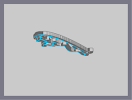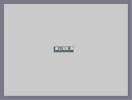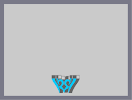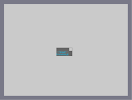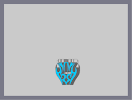Jaguar Maserati Logo C Ya Dragon Test One more Dragon Test

Pages: (3) [ 1 ] 2 3

### R3D_NINJ4

1. Paste map data into Microsoft Word (or similar program)
2. Have it do a count of all the times 8^ appears (for thumps) and how many times 1^ appears (for bounceblocks).

This map doesn't deserve any rating I can give it, but 5aved nonetheless.

### You sir,

are the definition of the Ultimate Supreme N God of Awesome Epicness.

### holy shit!

this is amazing. also how did you manage to count how many thwumps and blocks were in it?

### classic

i want this tattooed on my arm!

### i don't see the dragon either

but it is amazing, isn't it.

:S

### Clue:

The eyes of the dragon are not the holes visible.

### I'm not going to lie

I don't see the dragon. At all.

### Dude.

Whoever is bashing this clearly did not look at it. IT'S A DRAGON MADE OUT OF THWUMPS!!! AND IT! LOOKS! AWESOME!!!!!

### wahahaha

I love you eganic.

### What kinda review is this without a rating???

:(
Number 100
Great stuff.
This is so much more beautiful than the jaguar, but well, you know, beauty is in the eye

### I still preferred jaguar

but it's still amazing like hell.

.

### I still prefer the Jaguar

Although I can definately respect the work that went into this. Jaguar just seems more...finessed.

### ._________________.

I feel like this isn't old at all, and that I saw it yesterday.

Why.jpg

### WEIRD

I was looking for this map just yesterday after I saw you making it on YouTube!

### I remember this map.

Was on the top page for about 3 days.

### If someone had a computer fast enough to play this

It would be sickkk

### Any of his N-Arts.

Here's his user page. [nmaps.net]

### New compy

Just checking back this month.
Rhekatou:
I am on IRC all the time.
Lemme know what server or whatever its called and ill join ya.
Im already on one because i play Minecraft and use mIRC to keep logs.
Just lemme know.

1211:
I have see it and all i can say is, what is the picture of?
I can not see it whatever it is.

ftw

get on irc

### I rated 5/5 a while ago.

Sorry for the very late comment. It is one of my N-Arts on NUMA. Oh, and congrats on winning N-art of the Year. Have you ever heard of Dragon_Moon?

### WOOT!

I won the N-art of the year!
By a lot also.

Well, that is it.
I am gone from nmaps, but i still remember the days from when it was numa.notdot.net =D
Well, i will work on some more things on dan-ball's powder game, because i get better pictures there.
That is if i don't get too addicted to Minecraft.

I have a Deviant art account with the same name.
Check it out if you want, or not. Doesn't bother me either way.
Piratically ever where i have an account, it is the same name. (or very close).

But this is my last comment.
Hope y'all have a decent 2011.
and goodbye for quite sometime.
i will not be checking back regularly.

### CONEN

took you long enough to finish.

### autogen?

Never heard of it...
It is what i said it is. Me working on the map by hand the entire time, but speed up about 100 times.
If you don't believe me, i can upload all 18 hours of footage, but at 15 minute intervals it would take 74 videos on youtube.

During the video, i check for errors twice in the video. During those times, i used photoshop to find the errors.

Any other questions, i will try to answer as best as i can.

### you sound a little up yourself buddy

and looking at your time lapse, what the hell is that program? like Is this autogen? explain yourself
yes, there are not too many maps that are better than this.
Look at a full screen picture of those and mine will look better than theirs.

Kinda hard to tell if you are complementing my map.
Thanks...i think.

### Fact

I think the whole discussion of this is stupid. Here;s a fact IT'S level in a game on a computer, in a home, in a state, in a country, in a continent on an ocean in a planet, in a solar system, in a galaxy, in a universe, in the void. this is pathetic. Not to mention, this, took, time and work. There really inst much that is better than this, and that ,too is a fact.
I went looking through all of the narts recently and didn't see any of your stuff.
The dragon master i did, but nothing else.
And looking at it now, i cant see what its supposed to look like. (sry)

and the map listings mustn't be working right.
Just looking at yours and looked at 2 pages of 10 maps, found your zapdos, and went back to the 2 pages of 10 maps and there were different maps.

Im somewhat disappointed that this map didn't get over 100 votes and that it was knocked down to a 4/5 after about 35 votes.

And that jaguar that i made still had a mistake in it.
I think the only reason why it got such high a rating was that nothing had been made like it except one that had been deleted.
I make another like it and some people aren't impressed now.
=P

### RavenMac

I'm afraid there are at least 66 maps over 100 ratings, not to mention those between 85-100 not included. I made this list a long while back after my 180+ rates on my biggest nart... and it still does not come close to the best. You have a map on the list, your jaguar I believe, that beats even this. :P

But I have to agree that you are the only one that makes anything halfway decent with thwumps. ;)

### ok

Sorry for having suck a "large" image in the description.
Not like nobody else hasn't done it before.

@mahi_mahi
Id like to see you make a 5 minute map that's better than this.
Or anybody to make one.
And i looked last night, there aren't too many other n-art maps that are better than mine. (only 2 or 3)
Its just that i am the only recent person to make anything good with thwumps.

@squibbles
XD

@Geej15
Your being just as annoying as everyone else.

@miststalker06
RavenGod doesn't sound good to me. =P

Ok. I have to go to work now. So...bye

### This is fucking amazing.

This is pure awesomeness. Thank you for this map, RavenGod?

### @conen

nahhhhh....i dont think i will. especially since i did absolutely nothing to warrant that, ur just bein pissy. Again no need to be hostile

### Congrats. I'm neiher happy or sad that you've

finally been bumped, but personally I find it rude and obnoxious that people are voicing their satisfaction here. All of you should shut the buck up, we can all see that's it's bumped.
Anyway yes, congrats one more time.
Oh and lets see...
@Geej: Shut the fuck up.
@Luigi: Shut up, you sound dumb.
@Squibblies: I love you.